• 哈希表c++实现
千次阅读
2021-12-03 13:25:05

两大类，一类是结点的定义，一类是哈希表的定义：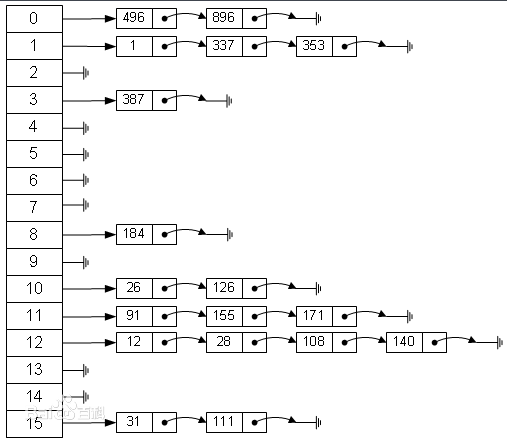具体的算法实现可以参考下面的程序：

.hpp文件

#pragma once
#include<iostream>
#include<ctype.h>

using namespace std;

typedef int Elemtype;
const int DEFAULT_SIZE=5;//如果没有指定桶的个数，就按默认值5

class Lnode
{
public:
bool IsEmpty()
{
if (this->next== nullptr)
return true;
else
return false;
}
public:
Lnode* next;
int key;
Elemtype data;
};

class Hash_Table
{

public:
Hash_Table();
int Hash_fun(Elemtype value);//定义哈希函数
void Create_HashTable(Elemtype* value, int size);//创建哈希表
bool unique_Lnode(Elemtype value); //判断结点是否重复
void Insert_Lnode(Elemtype value);//插入结点进入哈希表
void Debug();//测试一下
~Hash_Table();
private:
Lnode*table;
int num;
};

Hash_Table::Hash_Table()
{
this->num = DEFAULT_SIZE;
cout << "输入哈希桶的个数："; cin >>this-> num;
if (num <= 0)
this->num = DEFAULT_SIZE;
table = new Lnode[num];
for (int i = 0; i < this->num; i++)
{
this->table[i].data = NULL;
this->table[i].key=i;
this->table[i].next = nullptr;
}
}

int Hash_Table::Hash_fun(Elemtype value)
{
return (value % this->num);
}
void Hash_Table::Create_HashTable(Elemtype* value,int size)
{
for (int i = 0; i < size; i++)
{
int _key = this->Hash_fun(value[i]);
if (table[_key].IsEmpty())
{
Lnode* node = new Lnode;
node->data = value[i]; node->key = _key; node->next = nullptr; table[_key].next = node;
}
else//如果现在结点不为空，先去遍历，看是否该元素已经添加
{
bool reslut= this->unique_Lnode(value[i]);//reslut为1：该元素为新元素，为0，就不再添加了
if (reslut)
{
Lnode* node = new Lnode;
node->data = value[i]; node->key = _key; node->next = table[_key].next; table[_key].next = node;
}
else
continue;
}
}
}

bool Hash_Table::unique_Lnode(Elemtype value)//判断数据是否已经存在
{
int index = this->Hash_fun(value);
Lnode *temp = table[index].next;
while (temp)
{
if (temp->data == value)
return false;
temp = temp->next;
}
return true;
}

void Hash_Table::Insert_Lnode(Elemtype value)
{
int reslut = this->Hash_fun(value);
if (this->table[reslut].next==nullptr)
{
Lnode* node = new Lnode;
node->data = value; node->key = reslut; node->next = nullptr; table[reslut].next = node;
}
else//如果现在结点不为空，先去遍历，看是否该元素已经添加
{
bool reslut1 = this->unique_Lnode(value);//reslut为1：该元素为新元素，为0，就不再添加了
if (reslut1)
{
Lnode* node = new Lnode;
node->data = value; node->key = reslut; node->next = table[reslut].next; table[reslut].next = node;//前插法
}
else
return;
}
}

void Hash_Table::Debug()
{
cout << "测试如下：" << endl;
for (int j = 0; j < this->num; j++)
{
Lnode *temp = this->table[j].next;
cout << "索引为" << j << "的数值：";
if (temp == nullptr)
{
cout << "NULL" << endl;
continue;
}
while (temp)
{
cout << temp->data << "\t";
temp = temp->next;
if (!temp)
cout << endl;
}
}
}

Hash_Table::~Hash_Table()//尤其要注意开辟在堆区的数据的删除
{
for (int j = 0; j < this->num; j++)
{
Lnode* p1,*p2;
p1 = this->table[j].next;
p2 = p1;
while (p1)
{
p2 = p1->next;
delete p1;
p1 = p2;
}
this->table[j].next = nullptr;
}
delete[] this->table;
cout << "释放空间成功！" << endl;
}

main函数：

#include<iostream>
#include"哈希表.hpp"
using namespace std;

void text()
{
Elemtype ch[] = { 54,596,45,12,56,47,12,59,45,33,33,21,45,96,14,96,77,54,44,66,23,44 };
int length = sizeof(ch) / sizeof(ch);
Hash_Table H1;
H1.Create_HashTable(ch, length);
H1.Debug();
cout << "--------------------------------------" << endl;
H1.Insert_Lnode(888);
H1.Debug();
cout << "--------------------------------------" << endl;
}

int main()
{
text();
system("pause");
return 0;
}

测试的结果：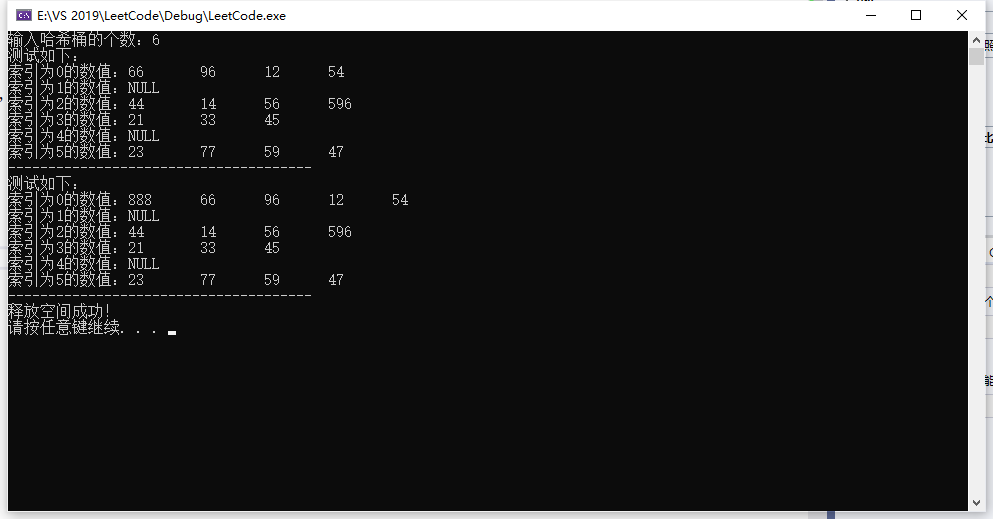### 讨论：

这里主要是针对int型建立的哈希表，哈希函数相对比较简单，对字符串等其他类型，需要改动程序。链表
更多相关内容
• 哈希表c++实现，数据结构课程资源，课上独立完成，供大家借鉴数据结构
• ## C++模拟实现哈希表

多人点赞 2022-05-22 19:22:20
C++模拟实现哈希表

# 🌲C++哈希

下面把放入哈希表中的值记为key，index是key在哈希表中的位置

## 🌴哈希冲突

两个key通过同一个映射关系得到了相同的值，即他们需要放在同一个位置（通过key算出的index一样），这就是哈希冲突。

简而言之，不同值映射到了同一个位置.

哈希表的效率与哈希冲突的处理密切相关

举个例子，哈希表大小为10，a[]={1，2，3，4，5，15}，定义哈希算法为 index=key%表长，从前往后构造哈希表。

即数组从前往后算出的index分别为1，2，3，4，5，5，此时key=15的元素算出的index为5，但是5这个位置已经有了key为5的元素，这就是哈希冲突

## 🌴负载因子

负载因子=哈希表中元素的个数/哈希表的大小

显然负载因子小于1，并且负载因子越大越容易产生哈希冲突（两个数放到一个位置的概率更大，大小为10的容器里放9个数冲突概率肯定比放一个数大）

## 🌴哈希函数

一般采用除留余数法，即取模的方法，如key对表长进行取模得到index。

此外还有很多种，如平方取中，随机数法等等

## 🌴闭散列

闭散列也叫开放地址法，发生哈希冲突时只要哈希表没满则说明哈希表中必有一个空位置存放这个值

### 🌵处理哈希冲突

线性探测和二次探测。

这两个方法都是发生了哈希冲突才用的上的.

#### 🌵线性探测

算出的index往后挨个找，找到一个空位置放入即可。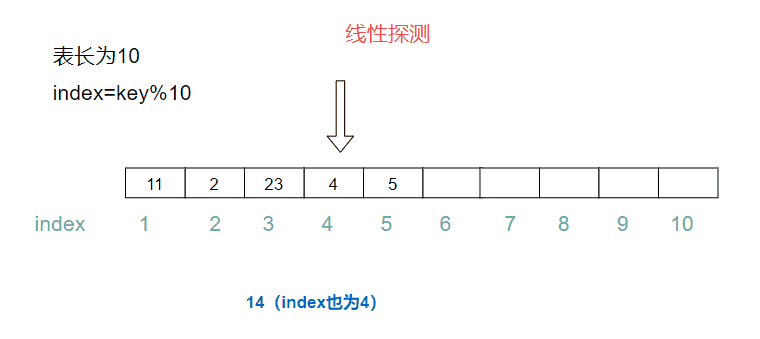#### 🌵二次探测

算出的index依次加1，4，9，…这种数，直到找到一个空位置

二次探测放入的数据更散，但是可能死循环（代码测试二次探测时一组数据死循环了）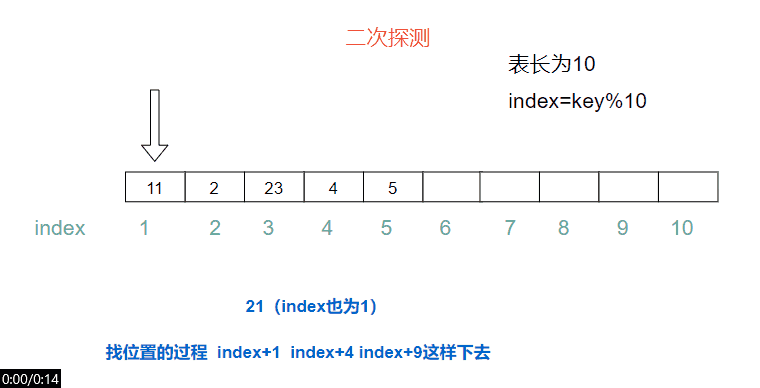#### 🌵其它

还有别的办法解决哈希冲突，闭散列主要用的上面两种，

## 🌴代码实现

### 🌵结点

template<class K, class V>
struct HashData
{
pair<K, V> _kv;
Status _status = EMPTY;
};


结点的三种状态

enum Status//定义一下结点的状态，方便操作
{
EMPTY,
EXIST,
DELETE
};


### 🌵内置类型以外类型的映射值处理

内置类型以外的类型就是自定义类型和库里的一些类型如pair和string等

我们利用仿函数得到相应的值

template<class K>
struct HashFunc
{
const K& operator()(const K& key)
{
return key;
}
};
template<>//对字符串进行特化，模板进阶的内容
struct HashFunc <string>
{
size_t operator()(const string& key)
{
size_t value = 0;
for (auto e : key)
{
value = value * 13 + (size_t)e;//乘以131是BKDR发明的字符串哈希算法，*131等数效率更高
}
return value;
}
};


仿函数传入key返回key有啥用？

以一般类型为例，如int，double等传进去直接返回它们本身的值，因为他们本身就是key。如STL中的set和unordered_set。

但是如果要传入pair呢？那就得我们自定义一个仿函数（如同处理string一样），可以写成下面这样

template<class K,class V>
struct HashFunc
{
const K& operator()(const pair<const K,V>& kv)
{
return kv.first;
}
};


仿函数写了如何用？传参时传入这个struct作为模板参数。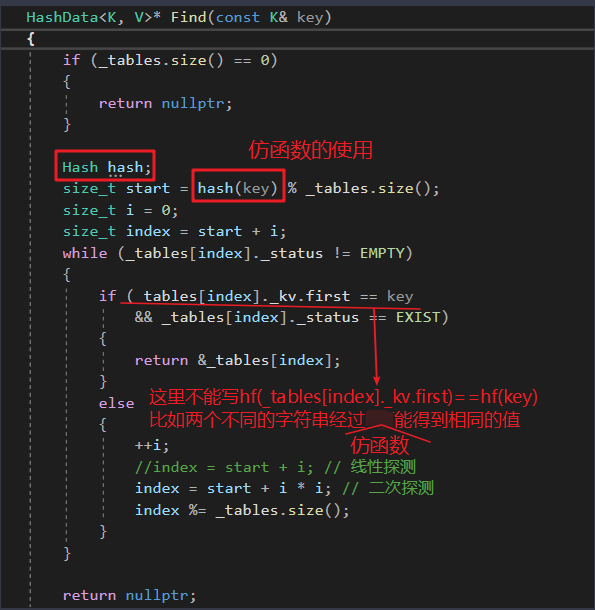### 🌵结构

	template<class K>
struct HashFunc
{
const K& operator()(const K& key) ;
};
template<>
struct HashFunc <string>
{
size_t operator()(const string& key);
};
enum Status//定义一下结点的状态，方便查找
{
EMPTY,
EXIST,
DELETE
};
template<class K, class V>
struct HashData
{
pair<K, V> _kv;
Status _status = EMPTY;
};
template<class K,class V,class Hash=HashFunc<K>>
class HashTable
{
public:
HashData<K, V>* Find(const K& key);
bool Insert(const pair<K, V>& kv);
bool Erase(const K& key);
private:
vector<HashData<K,V>>_tables;
size_t _n = 0;
};


### 🌵查找

思路：先算出key对应的index，如果这个位置存在且key与要查找的key相等就说明找到了，否则往后找，找到空的位置说明表中不存在这个key。

	HashData<K, V>* Find(const K& key)
{
if (_tables.size() == 0)
{
return nullptr;
}

Hash hash;
size_t start = hash(key) % _tables.size();
size_t i = 0;
size_t index = start + i;//算出的index
while (_tables[index]._status != EMPTY)//这个位置不为空
{
if (_tables[index]._kv.first == key
&& _tables[index]._status == EXIST)//存在且值相等
//判断是否存在是因为结点有一种可能的状态是删除
{
return &_tables[index];
}
else
{
++i;
//index = start + i; // 线性探测
index = start + i * i; // 二次探测
index %= _tables.size();
}
}

return nullptr;
}


### 🌵插入

思路：找到一个空位置直接放

具体来说，位置为空直接放，如果找到的位置已经有值了就通过线性探测/二次探测去后面找位置

值得注意的点是负载因子是0.7的时候或者刚开始表长度为0的时候得进行增容

增容拷贝原哈希表数据的方法：开一个新表，调用Insert函数插入数据到新表中，再交换新表和旧表即可。（vector的交换只交换指针，所以开销不大）

增容后表长变化，导致index也要跟着变，直接用新表插入就解决了这个问题

		bool Insert(const pair<K, V>& kv)
{
Hash hash;
if (Find(kv.first))//原来的表中存在
{
return false;
}
if (_tables.size()==0||_n*10/_tables.size()>=7)
//负载因子在0.7的时候扩容 不然可能导致死循环
{
size_t newsize = _tables.size() == 0 ? 10 : _tables.size() * 2;

HashTable<K, V, Hash>NewHT;//搞一个vector出来行吗？可以，但是选用hashtable后面可以直接调用Insert插入数据更方便
NewHT._tables.resize(newsize);
for (auto& e : _tables)//这种传引用 不传引用得多拷贝一次
{
NewHT.Insert(e._kv);
}
_tables.swap(NewHT._tables);//交换之后NewHT就被销毁了
}
size_t start = hash(kv.first) % _tables.size();
size_t i = 0;
size_t index = start + i;
//进行线性探测或二次探测
while (_tables[index]._status==EXIST)//不等于空就一直往后走
{
//index++;//由于负载因子在0.7时扩容了所以不会死循环.
i++;
//index = start + i 线性探测
index = start+i*i;
index %= _tables.size();
}
//找到位置了

_tables[index]._status = EXIST;
_tables[index]._kv = kv;
_n++;
return true;
}


### 🌵删除

复用Find()即可，这里是一种伪删除，只是更改结点状态而不是删除数据。

bool Erase(const K& key)
{

/*	Hash hs;
size_t index = hs(key) % _tables.size();
while (_tables[index]._status==EXIST)
{
if (_tables[index]._kv.first == key)
{
_tables[index]._status = DELETE;
return true;
}
index++;//线性探测
index %= _tables.size();
}
return false;*/
HashData<K, V>* ret = Find(key);
if (ret == nullptr)
{
return false;
}
else
{
ret->_status = DELETE;
return true;
}
}


### 🌵测试

测试整形

	void test1()
{
int a[] = { 5, 3, 100, 9999, 333, 14, 26, 34, 5};//这组数据在二次探测时会死循环
HashTable<int, int> ht;
for (auto e : a)
{
ht.Insert(make_pair(e, e));
}

ht.Erase(3);
cout << ht.Find(3) << endl;
}


测试字符串

void test2()
{
HashTable<string, string, HashFunc<string>>ht;
ht.Insert(make_pair("sort", "排序"));
ht.Insert(make_pair("entity", "实体"));
ht.Insert(make_pair("object", "对象"));
ht.Insert(make_pair("buffer", "缓冲"));
ht.Erase("sort");
cout << ht.Find("sort") << endl;
}


### 🌵析构，拷贝构造，构造函数

析构，结构选用了vector，vector会帮我们搞定

拷贝构造,没有涉及到深浅拷贝构造，vector会帮我们搞定

构造函数，给了缺省值，也没啥要传参初始化的情况，默认的也够用。

闭散列的哈希表可以理解为对vector进行了一次封装，vector的元素哈希结点也没开辟什么空间，所以也不会出现内存泄漏的问题，但是开散列采用链表的结构（链表是自己写的），所以需要自己去释放链表的空间。

## 🌴开散列（哈希桶）

STL里的unordered_set和unordered_map底层的哈希就选用了开散列结构

### 🌵处理哈希冲突

采用链表的形式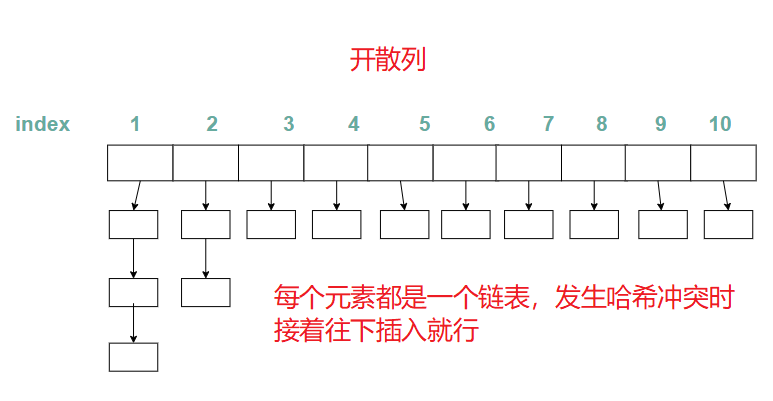• 那开散列的结构是不是不需要扩容？

需要的。以上图为例，表长就为10，我要放10000个数据，平均下来就算每个链表放1000个，那查找和删除指定元素的效率依旧不高。

• 那需要增容的话什么时候增容？

负载因子为1时增容

STL库里默认是1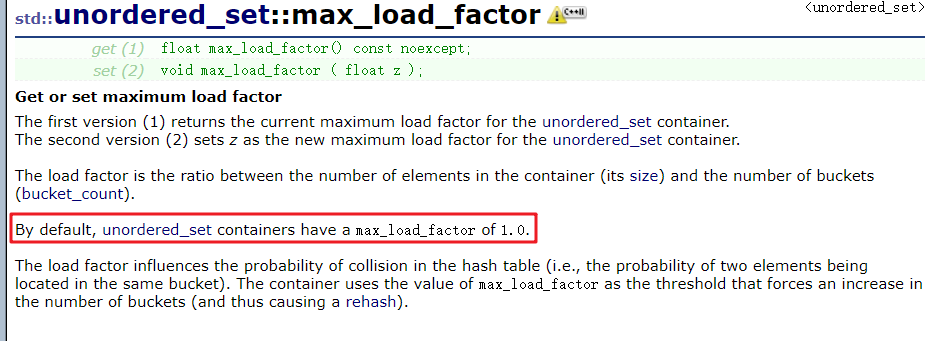## 🌴代码实现

#### 🌵结点

哈希表采用vector存储，vector的每个元素都是一个链表，链表的每个元素即是哈希结点

那么这个结点肯定需要有next指针指向下一个，又要存储类型的值，这里的类型采用pair<K,V>,所以就有了pair<K,V>

链表也可以用双向循环链表，单链表简单点，根据泛型编程的思想可以把pair改成模板。

struct HashNode
{
pair<K, V> _kv;
HashNode* _next;
HashNode(const pair<K,V>& kv)
:_kv(kv)
:next(nullptr)
{}
};


#### 🌵结构

namespace open_hash也行 开散列 ，但是命名空间取为哈希桶更加形象，每个桶都是一个链表

namespace HashBucket//防止与库里的一些名字相同导致命名冲突
{
template<class K,class V,class Hash=HashFunc<K>>
struct HashNode
{
pair<K, V> _kv;
HashNode* _next;
HashNode(const pair<K, V>& kv)
:_kv(kv)
: next(nullptr)
{}
};
template<class K,class V>
class HashTable
{
typedef HashNode<K,V> Node;
public:

Node* Find(const K& key)//Find函数返回值一般都是指针，通过指针访问这个自定义类型的成员
{

}
bool Insert(const pair<K, V>& kv)
{

}
bool Erase(const K& key)
{

}
~HashTable()//哈希桶采用的链表结构 需要释放每个链表
{

}
private:
vector<Node*>_tables;//存的是链表首元素的指针
size_t n;//有效数据
};

}


拷贝构造和赋值单重载后面独实现

#### 🌵查找

思想：先计算出桶号（对应的index），遍历桶号对应的这根链表，看是否能找到这个值

Node* Find(const K& key)//Find函数返回值一般都是指针，通过指针访问这个自定义类型的成员
{
Hash hash;//把string之类的类型映射成整数，即处理内置类型以外的类型
if (_tables.size() == 0)//表的大小为0，防止取余0
{
return nullptr;
}
size_t index = hash(key) % _tables.size();//找到桶号
Node* cur = _tables[index];
while (cur)
{
if (cur->_kv.first == key)
{
return cur;
}
else
{
cur = cur->_next;
}
}
return nullptr;
}


#### 🌵插入

思路：先去桶里找，去看看有没有这个值，如果有直接返回false。没有的话算出应该放入的桶，再将这个结点进行头插，插入前需要检查是否需要扩容。

为什么进行头插不进行尾插？因为尾插要找尾，由于采用的是单链表找尾得遍历这个链表更麻烦

怎么进行扩容？取出原来表的每个结点插入新表，再交换新旧两个表

注意扩容后表长变化导致index变化，比如原来表长是10，模10就行了，现在表长扩容成了20，就必须去模20了。同时一些本来在同一个桶的结点也可能不在同一个桶了。

bool Insert(const pair<K, V>& kv)
{
if (Find(kv.first))//有相同的key直接返回false
{
return false;
}
//if(_n==0||_n==_tables.size())
Hash hash;
if (_n == _tables.size())//最开始_n为0，而_tables.size()也为0所以可以简化为一行代码
//负载因子为1是增容
{
//增容
size_t newSize = _tables.size() == 0 ? 10 : _tables.size() * 2;
vector<Node*>newTables;
newTables.resize(newSize, nullptr);
for (int i = 0; i < _tables.size(); i++)
{
Node* cur = _tables[i];
while (cur)
{
Node* next = cur->_next;//记录下一个位置
size_t index = hash(cur->_kv.first) % newTables.size();
cur->_next = newTables[index];//cur当头
newTables[index] = cur;//更新vector里的头
cur = next;
}
}
_tables.swap(newTables);//把新表的数据放入旧表中
//思考newTable存在内存泄漏吗？
//newTable生命周期结束后调用自己的析构函数，又因为newTable交换后每条链表最多一个结点，所以默认的vector析构就够用。注意：交换后两个table都存了链表的头结点（通过调试可以清楚看到）
//原因：这里把旧表数据拷贝到新表其实就是单链表的拷贝，但是拷贝后旧表依然记住了一个结点（拷贝过程中没有操作旧表的vector，所以旧表vector存的Node*没变,不过头结点指针指向的Node里面的_next被置为空了，因为旧表每个桶的头结点拷贝过去都会是新表的最后一个结点（头插）），所以旧表拷贝前后都是记住了每个链表的头结点的，因此旧表每个桶最多只有一个头结点
}

size_t index = hash(kv.first) % _tables.size();//算出桶号
//头插
Node* newNode = new Node(kv);
newNode->_next = _tables[index];
_tables[index]=newNode;
++_n;//别忘记更新有效数据的个数
return true;
}


思考newTable存在内存泄漏吗？

newTable生命周期结束后调用自己的析构函数，newTable交换后每条链表最多一个结点，所以默认的结点的析构就够用。注意：交换后两个table都存了链表的头结点（通过调试可以清楚看到）

为啥newTable交换后每条链表最多一个结点？或者说拷贝后、交换前的旧表每条链表最多一个结点

原因：这里把旧表数据拷贝到新表其实就是单链表的拷贝，但是拷贝后旧表依然记住了一个结点（拷贝过程中没有操作旧表的vector，所以旧表vector存的Node*没变,不过头结点指针指向的Node里面的_next被置为空了，因为旧表每个桶的头结点拷贝过去都会是新表的最后一个结点（头插）），所以旧表拷贝前后都是记住了每个链表的头结点的，因此旧表每个桶最多只有一个头结点

插入时的效率变化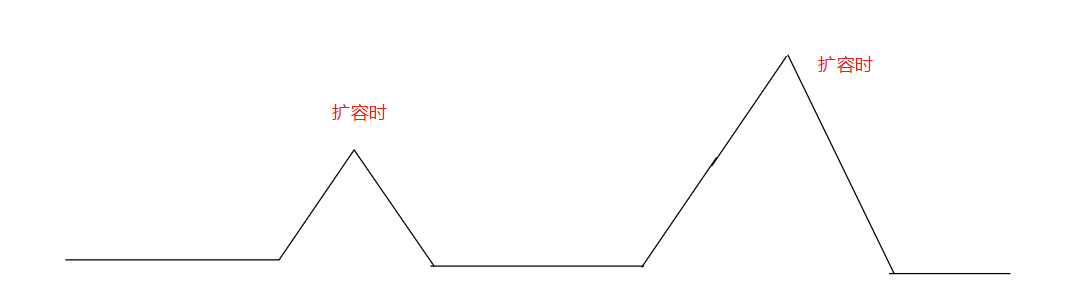#### 🌵删除

思路：先看这个元素存不存在，不存在直接返回false。存在的话算出桶号转为单链表的删除。

单链表的删除分为是否是头结点，先找到这个元素再看是不是头结点

为什么不直接Find()找到这个结点直接删除？单链表删除需要借助删除结点的前一个结点，Find只能找到当前结点。所以删除没有复用Find().

bool Erase(const K& key)
{
//if (!Find(key))//找不到这个元素
// 这么写也可以，但是后面删除的过程中会顺带遍历整个桶
//{
//	return false;
//}
if (_tables.size() == 0)//哈希表为空
{
return false;
}
Hash hash;
size_t index = hash(key) % _tables.size();
Node* cur = _tables[index];
Node* prev = nullptr;//记录前一个位置
while (cur)
{
if (cur->_kv.first == key)//找到这个元素了
{
if(cur==_tables[index])//元素是头结点
{
_tables[index] = cur->_next;

}
else//不是头结点
{
prev->_next = cur->_next;
}
delete cur;
cur = nullptr;
_n--;
return true;
}
else
{
prev = cur;
cur = cur->_next;
}
}
return false;
}


#### 🌵析构

判断桶的头结点是否为空，为空说明没有结点直接下一个，有的话从第二个开始遍历链表删除

为什么不从第一个删除

第一个的删除交给vector，如果从第一个开始会造成同一个元素析构两次，程序会崩…

~HashTable()//哈希桶采用的链表结构 需要释放每个链表
{
for (int i=0;i<_tables.size();i++)
{
Node* cur = _tables[i];
if (cur == nullptr)
{
continue;
}
else
{
cur = cur->_next;//不为空从第二个开始
}
while (cur)
{
Node* next = cur->_next;
delete cur;
cur = next;
}
_tables[i] = nullptr;
}
_n = 0;
}


#### 🌵拷贝构造和赋值重载

拷贝构造和赋值重载都要拷贝出完全相同的一份，所以需要尾插，由于单链表尾插需要找尾，效率不高。所以没有实现，网上找的资源也没找到答案。阅读STL源码的能力还不够，所以打算以后看源码怎么实现拷贝构造的。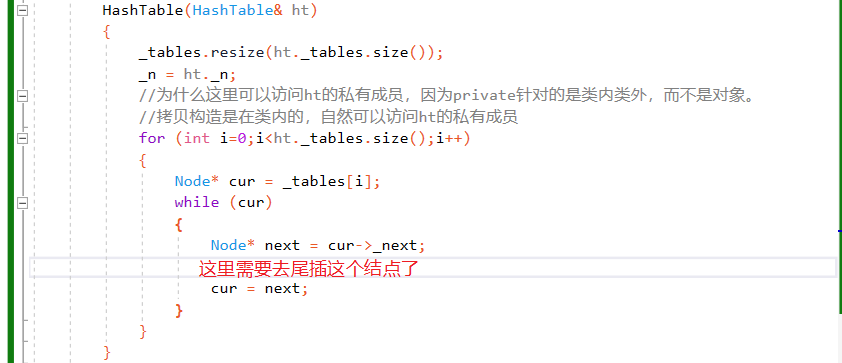#### 🌵优化

哈希表的大小建议是素数，网上有大佬可以探讨过了，这里直接贴一下网上的代码。

素数的话除留余数法时哈希冲突的可能性更小

		size_t GetNextPrime(size_t prime)
{
const int PRIMECOUNT = 28;
static const size_t primeList[PRIMECOUNT] =
{
53ul, 97ul, 193ul, 389ul, 769ul,
1543ul, 3079ul, 6151ul, 12289ul, 24593ul,
49157ul, 98317ul, 196613ul, 393241ul, 786433ul,
1572869ul, 3145739ul, 6291469ul, 12582917ul, 25165843ul,
50331653ul, 100663319ul, 201326611ul, 402653189ul, 805306457ul,
1610612741ul, 3221225473ul, 4294967291ul
};
//ul表示unsigned long
size_t i = 0;
for (; i < PRIMECOUNT; ++i)
{
if (primeList[i] > prime)
return primeList[i];
}

return primeList[i];
}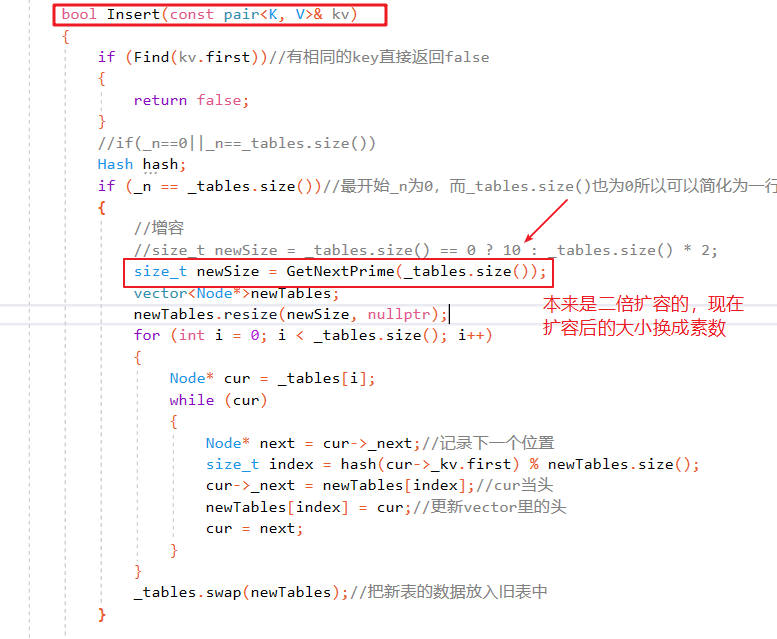## 🌴总结

哈希表的结构掌握后，处理哈希冲突即可。处理哈希冲突的方式不同会导致哈希表的效率不同。
github代码汇总

展开全文散列表 数据结构 c语言
• ## 哈希表、哈希桶（C++实现）

千次阅读 多人点赞 2022-01-23 19:41:13
哈希表、哈希桶哈希概念哈希函数哈希冲突解决哈希冲突闭散列线性探测闭散列的实现哈希表的结构哈希表的插入哈希表的查找哈希表的删除开散列哈希概念 哈希概念 在顺序结构和平衡树中，元素关键码与其存储位置之间没有...

# 哈希概念

在顺序结构和平衡树中，元素关键码与其存储位置之间没有对应的关系，因此在查找一个元素时，必须要经过关键码的多次比较。顺序查找时间复杂度为O(N)，平衡树中为树的高度，O(logN )，搜索的效率取决于搜索过程中元素的比较次数。

而理想的搜索方法：可以不经过任何比较，一次直接从表中得到要搜索的元素。 如果构造一种存储结构，通过某种函数(hashFunc)使元素的存储位置与它的关键码之间能够建立一一映射的关系，那么在查找通过该函数可以很快找到该元素。
向该结构中：

• 插入元素：根据待插入元素的关键码，以此函数计算出该元素的存储位置并按此位置进行存放
• 搜索元素：对元素的关键码进行同样的计算，把求得的函数值当做元素的存储位置，在结构中此位置取元素比较，若关键码相等，则搜索成功。
该方式为哈希(散列)方法，哈希方法中使用的转换函数称为哈希(散列)函数，构造出来的结构称为哈希表(Hash Table)(或者称散列表)

例如：数据集合{1,7,6,4,5,9}
哈希函数设置为：hash(key) = key % capacity; capacity为存储元素底层空间总的大小。
我们将数据映射到capacity为10的哈希表中如下：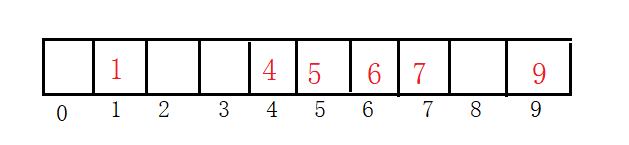这种方法的搜索速度很快。

# 哈希函数

常用的2种方法：

1.直接定址法：取关键字的某个线性函数为散列地址：Hash（Key）= A*Key + B

优点：简单、均匀
缺点：1需要知道关键字的分布情况，如果给的一组数据范围很大，就会浪费空间
2.不能处理浮点数，字符串等数据

2.除留余数法：设散列表中允许的地址数为m，取一个不大于m，但最接近或者等于m的质数p作为除数，按照哈希函数：Hash(key) = key% p(p<=m),将关键码转换成哈希地址

优点：数据范围很大
缺点：不同的值映射到同一个位置会冲突

# 哈希冲突

引起哈希冲突的一个原因可能是：哈希函数设计不够合理。 哈希函数设计原则：
1.哈希函数的定义域必须包括需要存储的全部关键码，而如果散列表允许有m个地址时，其值域必须在0 到m-1之间
2.哈希函数计算出来的地址能均匀分布在整个空间中
3.哈希函数应该比较简单

# 解决哈希冲突

## 闭散列-开放定址法

闭散列：也叫开放定址法，当发生哈希冲突时，如果哈希表未被装满，说明在哈希表中必然还有空位置，那么可以把key存放到冲突位置中的“下一个” 空位置中去。那如何寻找下一个空位置呢？

### 线性探测

线性探测：从发生冲突的位置开始，依次向后探测，直到寻找到下一个空位置为止。

Hash(key)=key%len+i(i=0,1,2…)

len：哈希表的大小
如果+0后面有数据那么再+1…直到找到空位置
比如插入11，和1冲突就往后探测。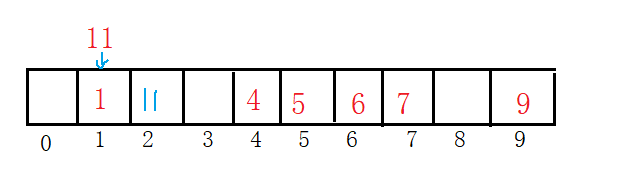动图演示：插入44通过上图发现：哈希表中的数据越多，产生哈希冲突的可能性会越大。此时我们引入了负载因子

负载因子=表中的有效数据/空间的大小

1.负载因子越大，产生冲突的概率越大，增删查改的效率低
2.负载因子越小，产生冲突的概率越小，增删查改的效率高

将哈希表的大小改为20在插入{1,7,6,4,5,9,11,13},产生的冲突变少：对于闭散列来说，负载因子是特别重要的因素，一般控制在0.7~0.8以下，超过0.8会导致在查表时CPU缓存不命中按照曲线上升。
线性探测的优点：实现简单
缺点：发生冲突，容易产生数据堆积，出现踩踏，导致搜索效率低。

✨✨✨✨✨✨✨✨✨✨✨✨我是分割线✨✨✨✨✨✨✨✨✨✨✨✨✨✨✨

二次探测

为了解决空位置是一个一个找，二次探测避免了这个问题。
二次探测不是再探测一次的意思，二次探测的方法

Hash(key)=key%len+i ^2(i=0,1,2…)

比如插入数据{1,4,5,9,11,13},为了测试我又加了44,54来看看效果：比之前的探测好了很多，之前的54是插在44后面的现在到了下表16了，避免了踩踏。

## 闭散列的实现

### 哈希表的结构

在闭散列哈希表中不仅要存数据之外，还要存储当前位置的状态，有3种状态：

1.EMPTY（无数据的空位置）
2.EXIST（已存储数据）
3.DELETE（原本有数据，但是被删除了）

我们用枚举即可

	enum State//标识每个位置的状态
{
EMPTY,
EXIST,
DELETE
};


为什么要加上状态？

那怎么标识一个空位置呢？用数字标识？例如1：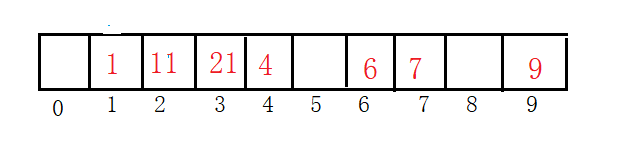但是把11删除后就找不到21了。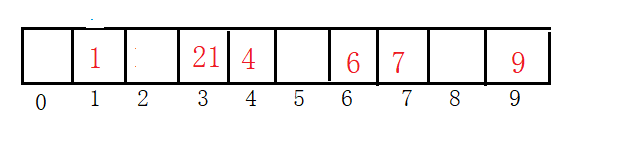它是和1冲突，从1开始找，1后面是空停止。但是21还在表中，不可能遍历一遍哈希表，这样就是去了哈希表的意义了。因此要在哈希表中加上状态。当哈希表中删除一个元素后，我们不应该简单的将状态设为EMPTY，要将该位置的状态设为DELETE。在查找的过程中，如果当前位置和查找的Key值不相等，但是当前位置的状态是EXIST或者是DELETE，我们都要往后查找，而当我们插入时，可以插入到状态EMPTY和DELETE上。

哈希表中的每个位置的存储结构包括状态和数据

	template<class K, class V>
struct HashDate
{
pair<K, V> _kv;//数据
State _state = EMPTY;//状态
};


我们还要在哈希表中存入数据的个数来计算负载因子，当负载因子过大就要将哈希表增容。

template<class K, class V>
class HashTable
{
public:
//...
private:
vector<HashDate<K, V>> _table;
size_t _n = 0;//哈希表中元素的个数
};


### 哈希表的插入

插入的逻辑如下：
1.查看是否存在该键值对，如存在则返回false
2.判断哈希表的大小是否需要调整，为0或者是负载因子过大都需要调整
3.插入元素
4.哈希表大小加1

增容的逻辑：
不能原地扩容，那原来数据的映射关系就乱了。我们要新创建一个哈希表对象，是原来的2倍，把旧的数据插入到新表中，在交换2张表即可。

插入键值对的过程：
1.先计算出对应的位置
2.如果发生冲突，进行线性探测处理，向后找到一个状态为EMPTY或者DELETE的位置插入
3.插入到该位置，并将状态设为EXIST

代码如下：

bool insert(const pair<K, V>& kv)
{
//通过查找看看哈希表中是否存在
HashDate<K,V>* ret = Find(kv.first);
if (ret)
{
//如果存在返回false
return false;
}
if (_table.size() == 0)
{
_table.resize(10);
}
else if ((double)_n /(double)_table.size() > 0.7)
{
//增容
HashTable<K,V> newHT;//创建一个新的表
newHT._table.resize(2 * _table.size());
//将旧表的数据插入到新的哈希表
for (auto& e : _table)
{
if (e._state == EXIST)
{
newHT.insert(e._kv);
}
}
_table.swap(newHT._table);
}
//计算位置
size_t start = kv.first % _table.size();
size_t index = start;
size_t i = 1;
while (_table[index]._state == EXIST)
{
index = start + i;//线性探测
index %= _table.size();//防止超出表的范围
++i;
}
//找到可以插入的位置
_table[index]._kv = kv;
_table[index]._state = EXIST;//调整状态
_n++;//哈希表元素++
return true;
}


### 哈希表的查找

查找的步骤：
1.先判断哈希表是否为0，等于0返回false，因为不能除0操作
2.除留余数算出映射位置
3.从映射的地址开始线性探测查找，找到返回成功，找到一个状态为EMPTY返回false

重点：在查找时，必须找到位置状态为EXIST且Key值相等，才能查找成功。仅仅是Key值相等，但是当前位置的状态为DELETE，则还需要继续向后查找，因为该位置的值已经删除了。

代码如下：

		HashDate<K,V>* Find(const K& key)
{
//表为空返回false
if (_table.size() == 0)
{
return nullptr;
}
size_t start = key % _table.size();//计算出映射的地址
size_t index = start;
size_t i = 1;
while (_table[index]._state != EMPTY)
{
//该位置的状态为EXIST，且Key值相等，查找成功
if (_table[index]._state == EXIST
&& _table[index]._kv.first == key)
{
return &_table[index];
}
//继续线性探测查找
index = start + i;
index %= _table.size();
i++;
}
return nullptr;
}


### 哈希表的删除

删除的过程很简单，我们只需要进行伪删除，将待删除元素的状态设为DELETE即可。
步骤如下：

1.先调用查找，找到该元素，若没有找到返回false
2.找到将状态设为DELETE
3.哈希表中的有效数据–

代码如下：

	bool Erase(const K& key)
{
HashDate<K, V>* ret = Find(key);
if (ret)
{
ret->_state = DELETE;
--_n;
return true;
}
return false;
}


下面来测试测试：

void Test_Hash()
{
int a[] = { 1,5,10,1000,100,18,15,7,40 };
CloseHash::HashTable<int, int> ht;
for (auto e : a)
{
ht.insert(make_pair(e,e));
}
auto ret = ht.Find(10);
if (ret)
cout << "找到了" << endl;
else
cout << "没找到" << endl;
ht.Erase(10);
ret = ht.Find(100);
if (ret)
cout << "找到了" << endl;
else
cout << "没找到" << endl;
ret = ht.Find(10);
if (ret)
cout << "找到了" << endl;
else
cout << "没找到" << endl;
}


我先找10，在删除10，看看能不能找到100，在看看10删除后能不能找到。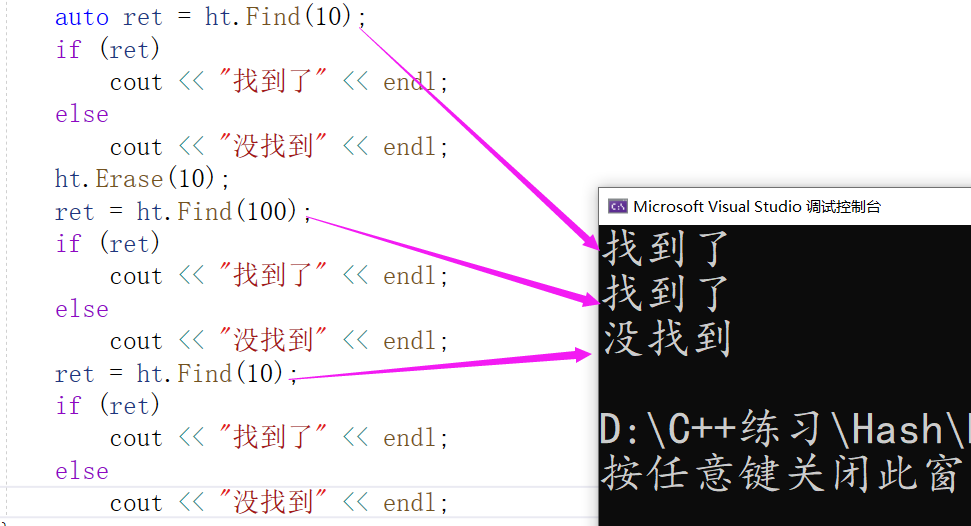是没有问题的。

## 开散列的实现-拉链法

开散列法又叫链地址法(开链法)，首先对关键码集合用散列函数计算散列地址，具有相同地址的关键码归于同一子集合，每一个子集合称为一个桶，各个桶中的元素通过一个单链表链接起来，各链表的头结点存储在哈希表中。
例如：下面的数据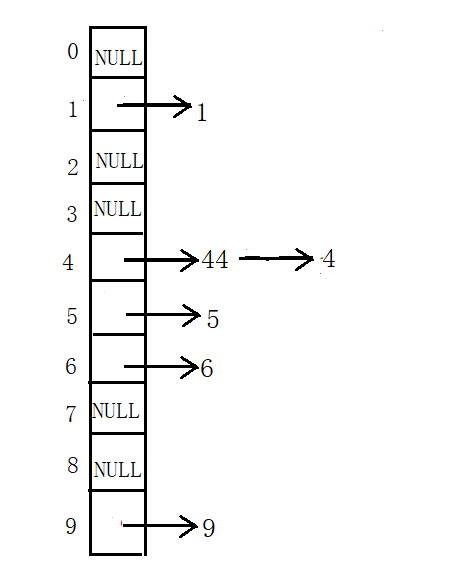动图演示：✨✨✨✨✨✨✨✨✨✨✨✨我是分割线✨✨✨✨✨✨✨✨✨✨✨✨✨✨✨

### 哈希表的结构

哈希表的每个位置存某个单链表的头结点，所以每个哈希桶中存储的数据应该是一个结点的类型，还需要一个节点的指针指向它的下一个结点。

	template<class K,class V>
struct HashNode
{
HashNode<K, V>* _next;//结点的指针
pair<K, V> _kv;//键值对
//构造函数
HashNode(const pair<K,V> kv)
:_next(nullptr)
,_kv(kv)
{}
};
template<class K, class V>
class HashTable
{
public:
typedef HashNode<K,V> Node;
private:
vector<Node*> _table;//哈希表
size_t _n = 0;//缺省值
};


### 哈希表的插入

插入的步骤：
1.它是不允许冗余的，所以先查找Key,存在返回false
2.检查负载因子，如果哈希表为空或者负载因子过大就需要进行增容处理
3.计算出在哈希表的位置进行头插
4.哈希表的有效数据在++

增容处理：

1.创建新的哈希表，大小是原来旧表的2倍
2.遍历旧表，算出在新表中的位置，在将结点头插到新表中
3.旧表位置的指针置空，交换2张哈希表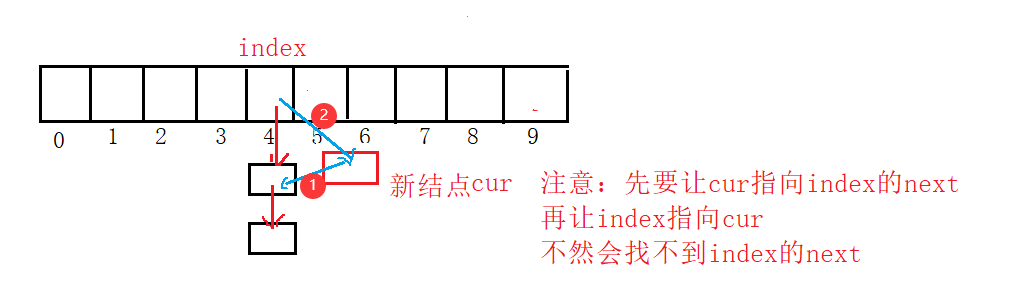代码如下：

//插入
bool insert(const pair<K,V>& kv)
{
//如果表中已经有该元素返回false,不能出现数据冗余
HashNode<K, V>* ret = Find(kv.first);
if (ret)
{
return false;
}
//检查负载因子,负载因子超过1或者table.szie=0需要调整
if (_n == _table.size())
{
//进行增容,创建一个新的哈希表
vector<Node*> newtable;
//新表的大小等于旧表的2倍
size_t newsize = _table.size() == 0 ? 10 : _table.size() * 2;

newtable.resize(newsize, nullptr);//把newsize给新表，并将表的位置初始化
//把旧表的结点插入到新表中
for (size_t i = 0; i < _table.size(); ++i)
{
if (_table[i])//桶不为空,插到新表中
{
Node* cur = _table[i];
while (cur)//桶中的结点不为空
{
//计算新表中的映射位置
size_t index = kv.first % newtable.size();
//保存cur的下1个结点，不保存头插的话找不到下个结点
Node* next = cur->_next;
cur->_next = newtable[index];
newtable[index] = cur;

cur = next;
}
_table[i] = nullptr;//旧桶置空
}
}
_table.swap(newtable);//交换2个哈希表
}
//计算出映射的位置
size_t index = kv.first % _table.size();
Node* newnode = new Node(kv);
//头插
newnode->_next = _table[index];
_table[index] = newnode;
++_n;

return true;
}


### 哈希表的查找

查找的步骤：
1.计算出在哈希表中的映射位置
2.遍历结点在链表的位置
注意：哈希表为0时处理一下，不能进行除0操作

		//查找
HashNode<K, V>* Find(const K& key)
{
if (_table.size() == 0)
{
return nullptr;
}
//1.先计算出映射的位置
size_t index = key % _table.size();
//2.遍历链表的位置
Node* cur = _table[index];
while (cur)
{
//找到返回结点
if (cur->_kv.first == key)
{
return cur;
}
else
{
cur = cur->_next;
}
}
//没找到返回空
return nullptr;
}


### 哈希表的删除

删除的步骤：
1.还是先算出在哈希表中的映射位置
2.开始删除
3.哈希表中的数据–
删除的时候注意删除第1个结点

	//删除
bool Erase(const K& key)
{
//1.先计算出映射的位置
size_t index = key % _table.size();
//2.开始删除
Node* cur = _table[index];
Node* prev = nullptr;//保存cur的前1个结点
while (cur)
{
//找到了开始删除
if (cur->_kv.first == key)
{
if (prev == nullptr)//删除第1个结点
{
_table[index] = cur->_next;//指向cur的下一个结点
}
else
{
prev->_next = cur->_next;
}
delete cur;//释放该结点
_n--;//哈希表中数据--
return true;
}
prev = cur;
cur = cur->_next;
}
return false;//没有该结点返回false
}


下面来测试一下，博主这里是没有实现迭代器的。等到封装unordered_set和unordered_map的时候在实现迭代器。

void Test_Hash1()
{
int a[] = { 1,5,10,20,18,15,7,40 };
OpenHash::HashTable<int,int> ht1;
for (auto e : a)
{
ht1.insert(make_pair(e, e));
}
auto ret = ht1.Find(10);
if (ret)
cout << "找到了" << endl;
else
cout << "没找到" << endl;
ht1.Erase(10);
ret = ht1.Find(20);
if (ret)
cout << "找到了" << endl;
else
cout << "没找到" << endl;
ret = ht1.Find(10);
if (ret)
cout << "找到了" << endl;
else
cout << "没找到" << endl;
}


插入好的效果如下图：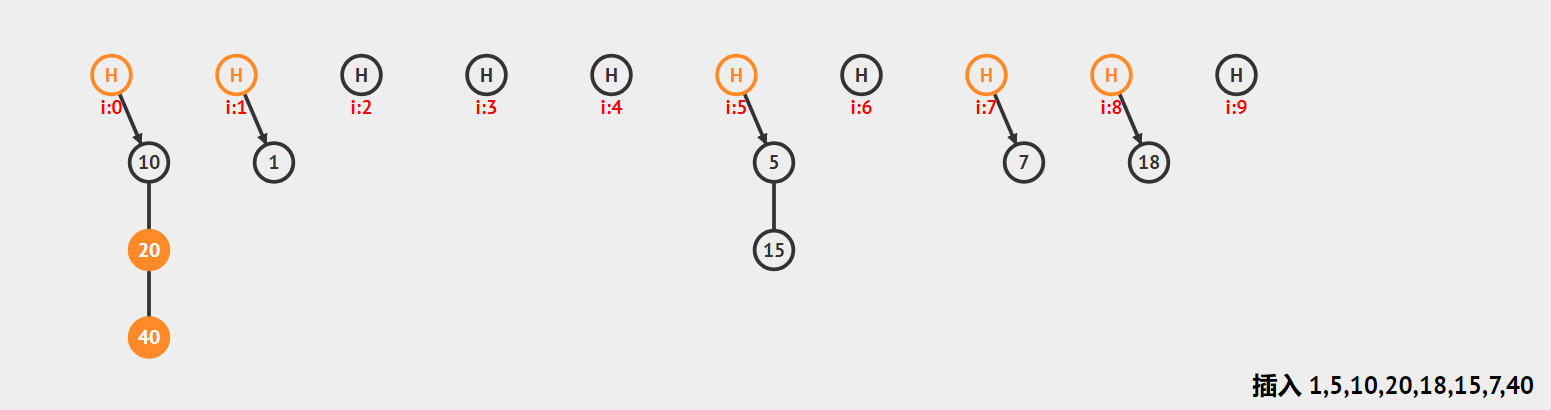我们通过调试来看看：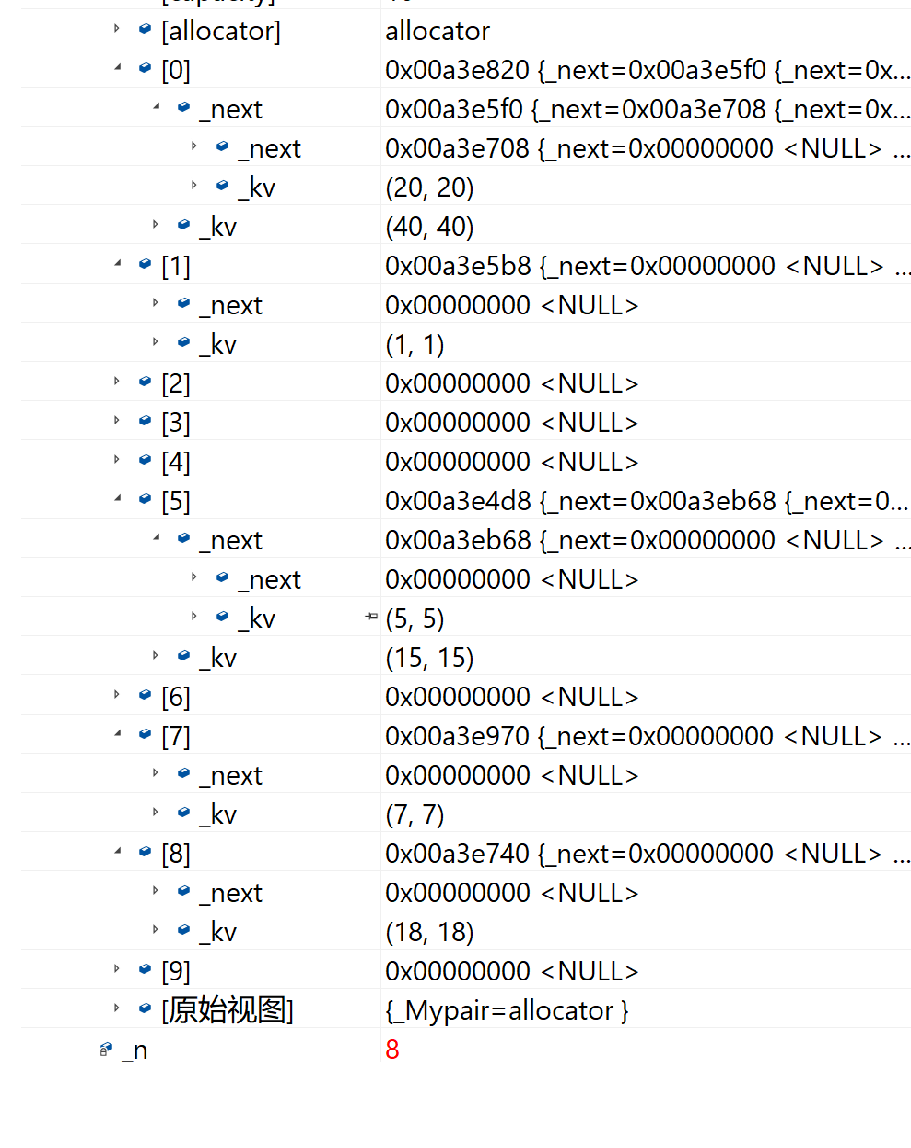看出是没有问题的。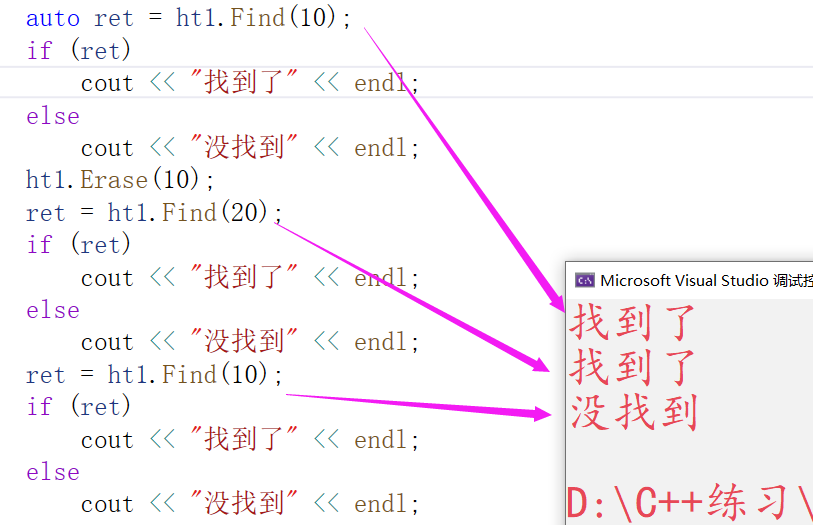查找也都可以。

# 小结

在实际中哈希桶更实用，在极端的情况下也能处理。比如下图，在下面挂了很多的结点，此时效率就会很低很低。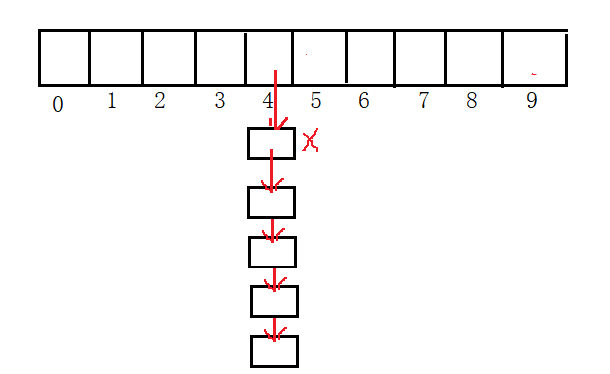前辈们的办法是把挂多的结点放在红黑树中。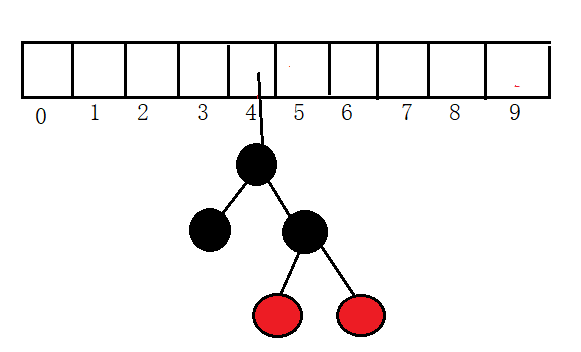桶中种树所以前辈还是很牛的。在JAVA中比较新的版本中结点超过8个就会将单链表改成红黑树，结点少于8个又会变回单链表的结构。这种结构我们知道即可，不用我们自己去实现。
但是有的地方没做这种处理也是可以的，当负载因子够大的时候就会触发增容的机制，就会开更大的表，映射位置也就随之改变而桶中的结点也会减少。

展开全文散列表
• 模拟实现哈希表两万字详解，包括哈希表的闭散列实现和开散列实现，以及迭代器的实现😇 😇hello！我是bug。前面几期我们讲解了树的相关结构，今天我们就要进入哈希表的学习了：
（代码可能会有一点问题，请各位老铁指正 😘 😘 ）

# 一、哈希

🌵🌵在前面，我们学习了红黑树、AVL树的相关性质，他们增删查改的时间复杂度都是O（logN），这个效率已经是比较高的了。但是有没有一种数据结构，（不需要进行比较就可以直接增删查改）它进行增删查改的时间复杂度为O（1） 呢？下面我们就要介绍这种数据结构——哈希表。

## 🍉 1、什么是哈希？

🌵🌵哈希（Hash）散列函数，其本质是映射

🌻 🌻那么映射又是什么呢？如下图：（例如医院的挂号系统）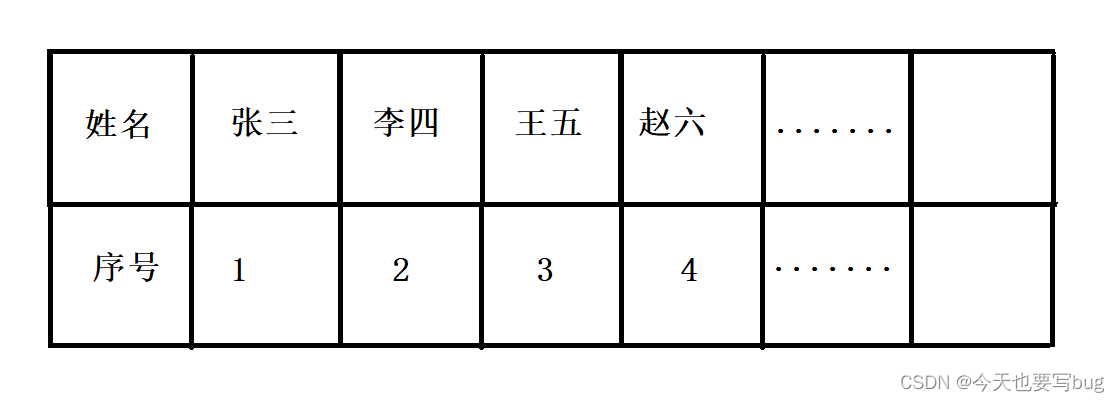其中我们存入一对键值，通过序号我们就可以找到人员。而序号和姓名之间就是一种映射关系。（也就是KV模型）

🌵🌵 哈希表（HashTable）是根据关键码值(Key value)而直接进行访问的数据结构。通过把关键码值映射到表中一个位置来访问记录，以加快查找的速度。这个映射函数叫做散列函数，存放记录的数组叫做散列表。
通过这种映射关系，那么我们就可以直接通过key值直接找的相应的数据，插入和删除数据时也可以直接根据key值进行插入删除，其时间复杂度就是O（1） 。

🌵🌵作用哈希表在增删查改上的效率都是十分优秀的，一般我们都是通过哈希表来快速查找数据是否出现在表中。

🌵🌵哈希冲突在绝大多数情况下，我们都会面临一个问题，那就是如何使一个位置映射一个数据。当我们开辟一个数组时，我们是采用下标来进行映射的，即每一个下标都会映射数据。但是当多个数据都映射到同一个位置时，那么就产生了哈希冲突。对于哈希冲突我们是要尽量避免的，因为哈希冲突会降低我们增删查改的效率。（具体原因下面再介绍）

🌵🌵哈希表的K模型和KV模型和前面的红黑树（AVL树）一样，所以哈希表也可以封装map和set。C++中的unordered_map和unordered_set的底层就是哈希表。

🌵🌵缺点当我们要对大量数据进行操作时，要开辟大量的空间来存储数据。而且，散列函数的采用也是至关重要的（尽量避免哈希冲突），那么我们要采取哪种散列函数来进行映射呢？（下面进行介绍）

## 🍉 2、直接定址法

假设有这样一个数组，我们要将它存入哈希表中：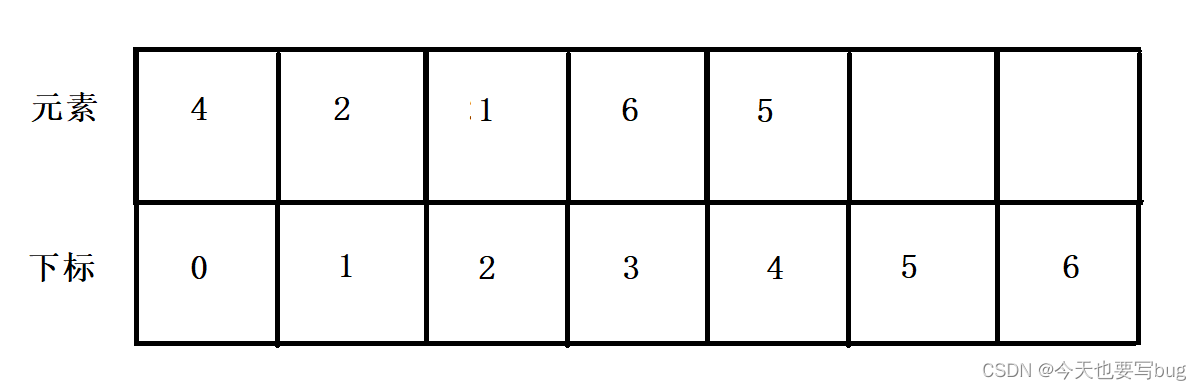我们直接根据元素的实际大小进行插入。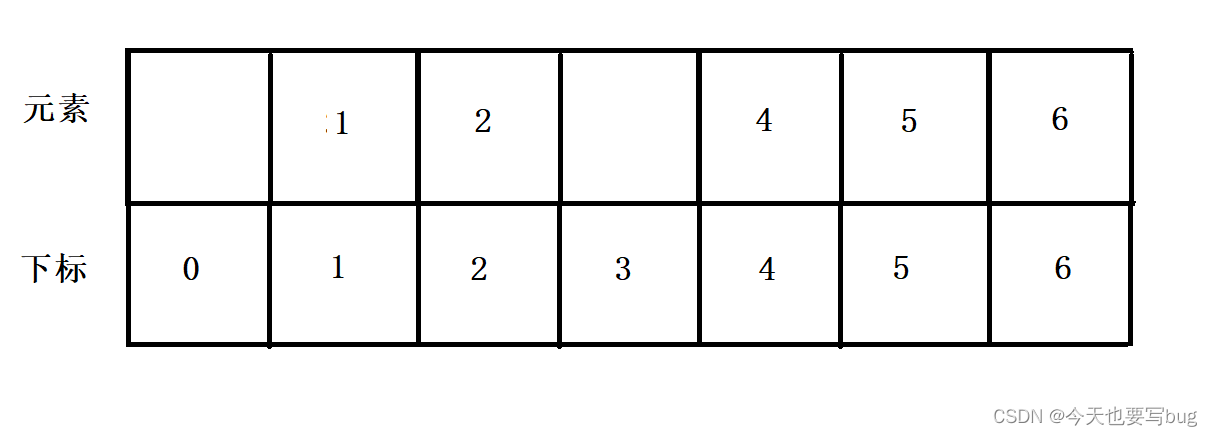如上图，即每一个下标都对应一个相应的数据，没有对应的数据就为空

🍏 注意 ❗️ ❗️

🌳🌳 （1）开辟数组的时候，一定要知道数据的范围，并根据数据的范围开辟数组，如果数据的大小超过了数组的大小就会越界。
🌳🌳（2）采用直接定址法一定要数据比较集中，因为最大最小数据如果相差太大，那么浪费的空间也会很多。
🌳🌳（3）如果我们存储的是整型，那么不需要担心哈希冲突，因为每个数值都对应唯一的位置。

🌻 🌻但是，当我们存入的数据不是整型的时候我们就要考虑如何来进行映射？
🌻 🌻其实在大多数情况下，我们采用unordered_map和unordered_set存储的都是字符串，那我们如何将字符串和下标联系起来呢？

可能你会觉得字符都有对应的ASCII码，我们可以用字符的ASCII来进行对应。但是这种情况下就不可避免的会出现哈希冲突，因为只要我们不规定字符串的长度，那么字符串就是无穷无尽的，通过其ASCII来对应是一定会产生冲突的。（对于哈希冲突的解决我们下面再来进行详细的介绍）

## 🍉3、除留余数法（重点）

当一组数据中的最大和最小相差比较大时，就会浪费大量的空间。所以直接定址法就不再适用了，这时候我们就要采用除留余数法。

🌻 🌻什么是除留余数法呢？
假设有这样一个数组，如下图：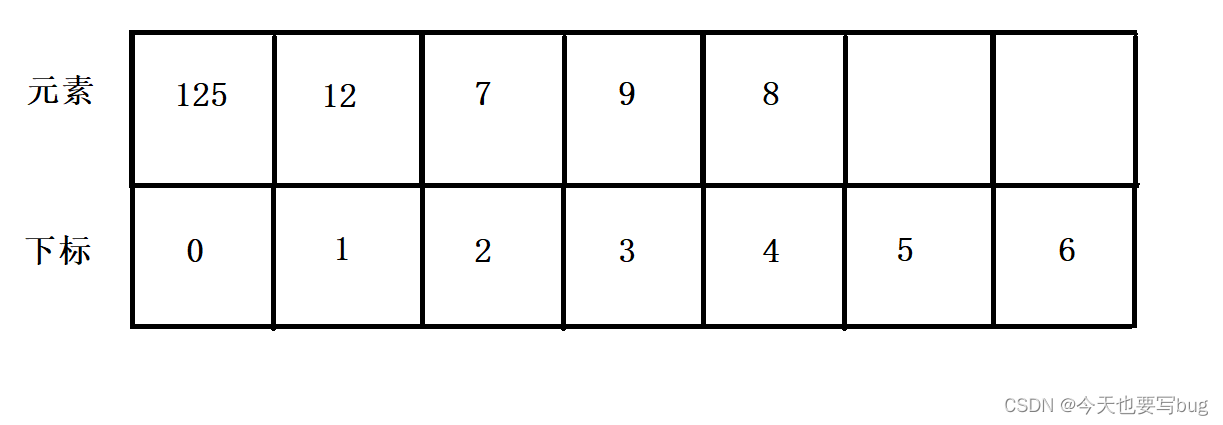🌻 🌻如何通过除留余数法将数据存入哈希表中呢？

即我们将每一个数据对表的大小进行取模，取模过后数据就可以通过下标来映射相应的位置。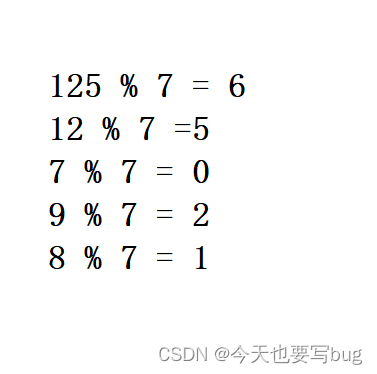所以插入后数据如图：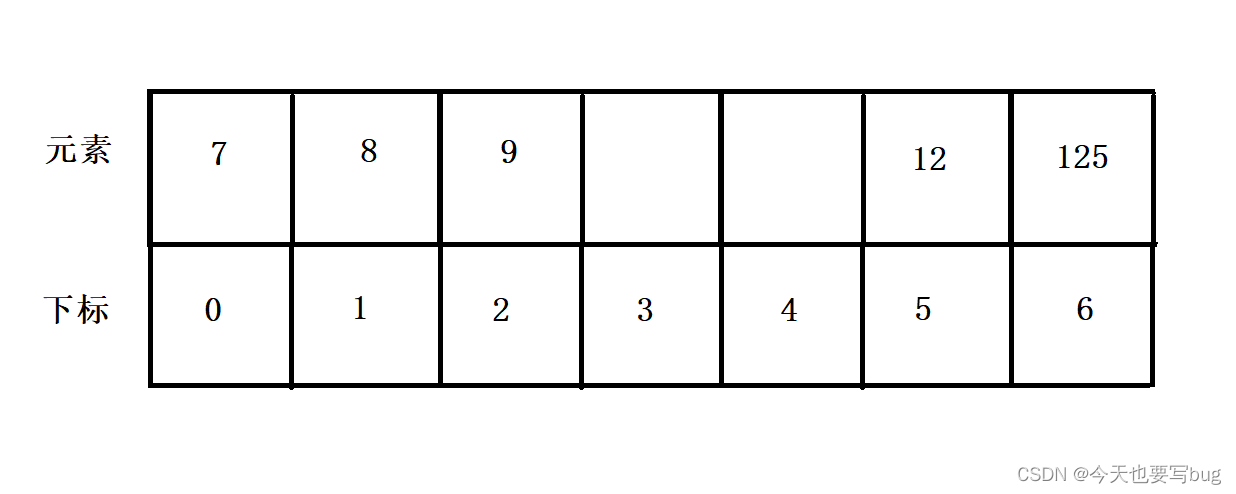除留余数法可以很好的避免空间大量的浪费，所以一般我们都采用除留余数法。当然，除留余数法也会产生哈希冲突。

除了直接定址法和除留余数法，我们还有其他的方法比如：平方取中法、折叠法、随机数法、数学分析法。（但是这些方法不常用，这里不做介绍）

🍏 注意❗️ ❗️

🌳🌳 哈希函数设计的越精妙，产生哈希冲突的可能性就越低，但是无法避免哈希冲突。🍓 🍓 下面就是实现重点了，嘿嘿。

## 🍉4、解决哈希冲突

上面直接定址法和除留余数法都会产生哈希冲突的问题，下面我们就来解决：

### 🌱 闭散列

🌵🌵闭散列也叫做开放定址法，当发生哈希冲突时，如果哈希表未被装满，说明在哈希表中必然还有空位置，那么可以把key存放到冲突位置中的“下一个” 空位置中去。那如何寻找下一个空位置呢？

#### 🍄 （1） 线性探测

🌵🌵线性探测 线性探测是计算机程序解决哈希表冲突时所采取的一种策略。

还是这个数组：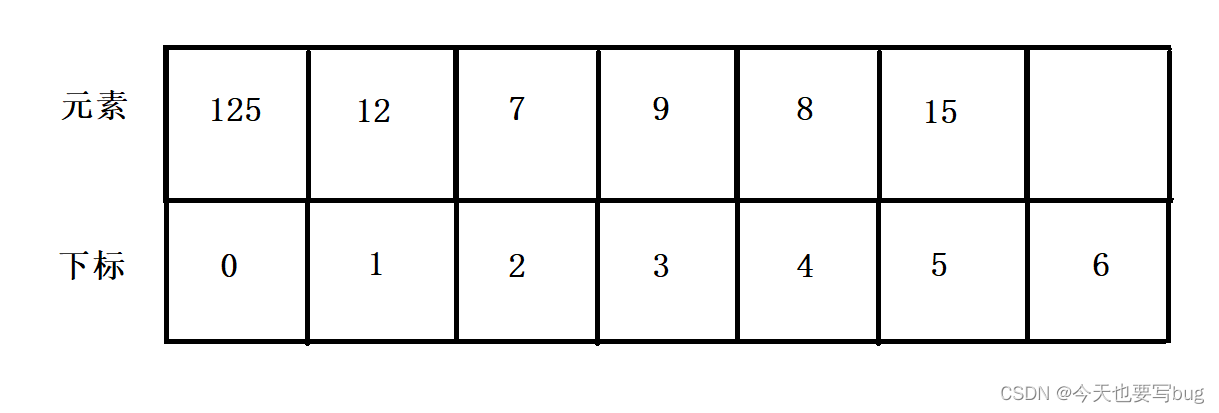我们通过除留余数法，可以算得8和15取模后，其对应同一个位置，那么我们从这个位置开始，一个一个往后面去探测，直到找到一个空位置，就将数据插入。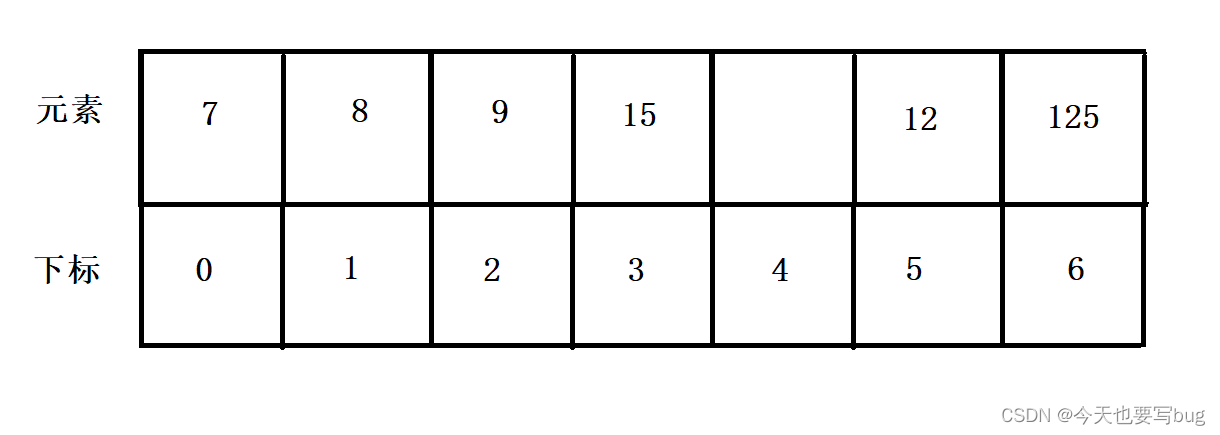但是会有这种情况，即：取模后发生冲突，然后向后面探测，直到探测到最后一个位置都没有发现空位置，那么我们就再从头开始进行探测。

（线性探测中探测的终点就是取模后对应的位置，因为当我们从开始取模位置探测，探测到最后一个位置都没有，那么我们再从头开始探测，如果还是没有，那么会又回到取模位置。所以我们表的大小一定要超过数据的个数。）

🍐 优点 十分简单，好操作。

🍐 缺点 会造成成片冲突。一旦发生哈希冲突，所有的冲突连在一起，容易产生数据“堆积”。（如下图）

如下数组：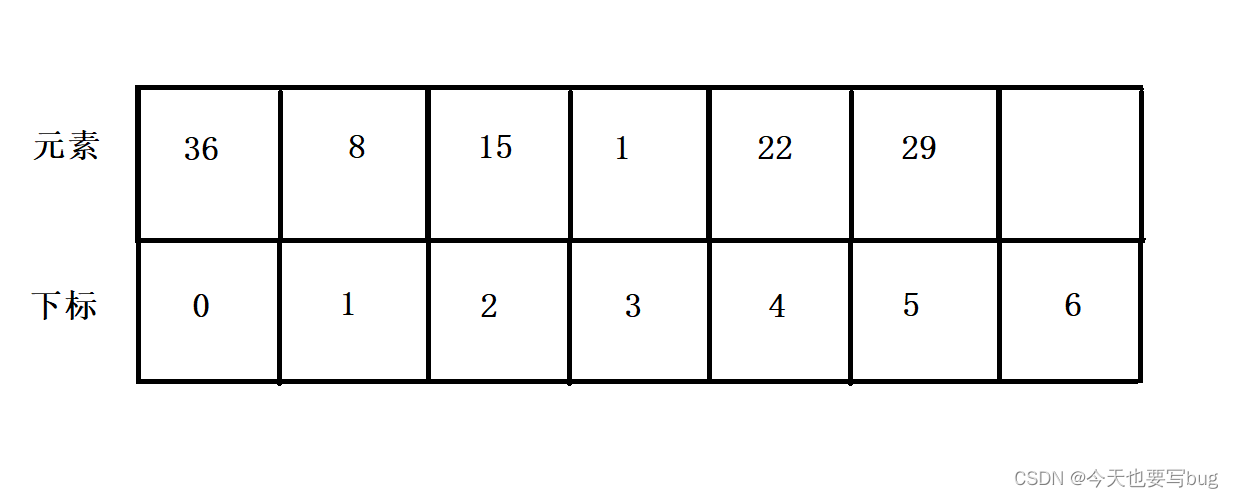其中每个数据取模后都对应下标为1的位置，通过线性探测插入后，如下图：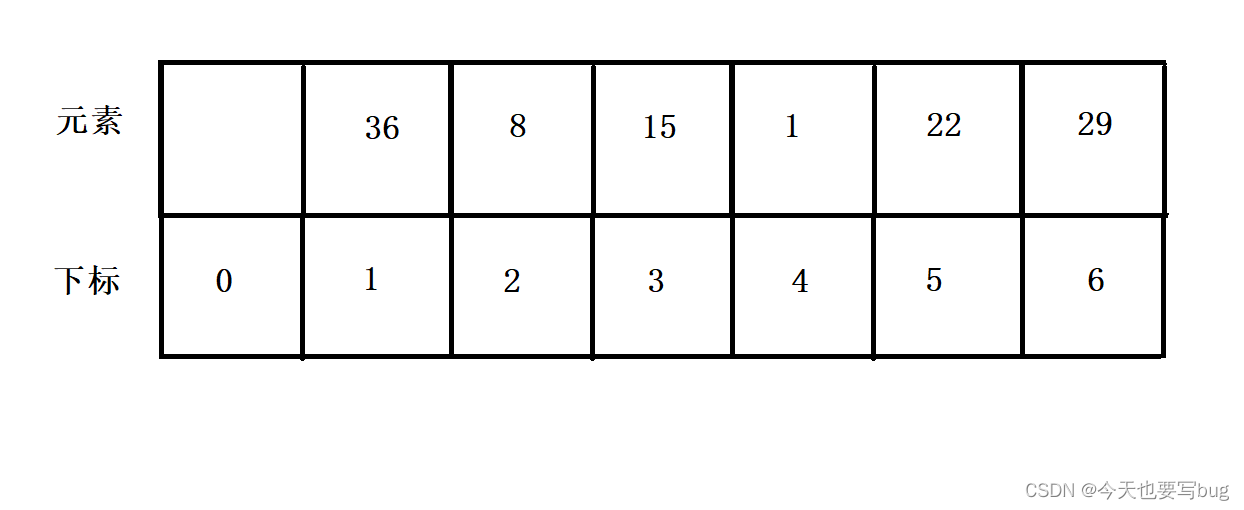当我们进行删除查找的时候，都是通过取模找到位置1，如果位置1不是我们要查找的数据，就会向后面遍历，直到找到数据为止。这也是为什么哈希冲突的发生会造成效率下降，而当大量的哈希冲突扎堆时，其效率更会大幅度下降。所以，也就引出了下面的二次探测来缓解成堆的哈希冲突。

#### 🍄 （2） 二次探测

🌵🌵二次探测线性探测的缺陷是产生冲突的数据堆积在一块，这与其找下一空位置有关系，因为找空位置的方式就是挨着往后逐个去找，因此二次探测为了避免该问题，找下一个空位置的方法为： = ( + )% m,或者： = ( - )% m。其中：i = 1,2,3…， 是通过散列函数Hash(x)对元素的关键码 key 进行计算得到的位置，m是表的大小。

还是看图，有这样一组数据：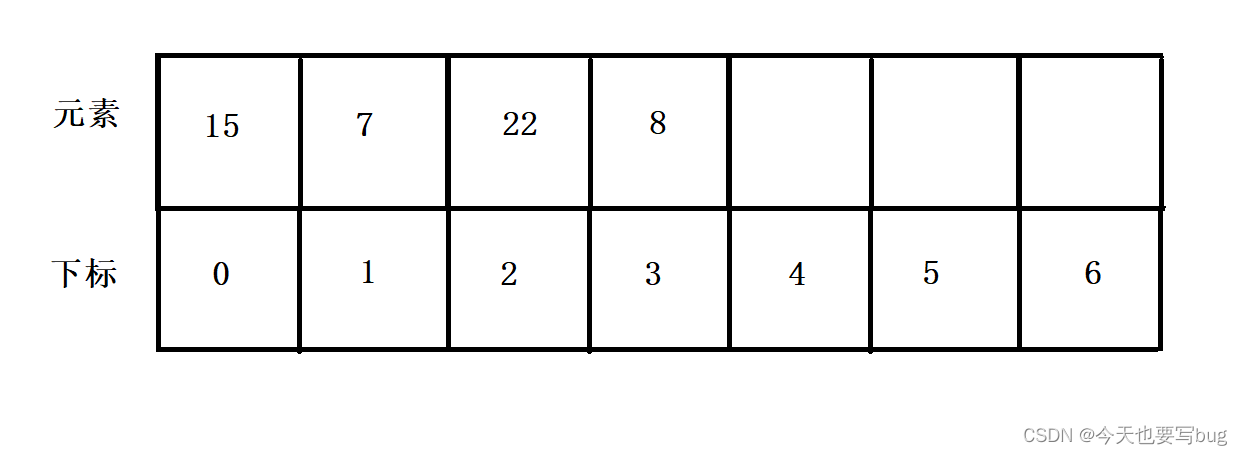通过二次探测后，即15、22和8都对应位置1，先插入15，接着我们插入22时，二次探测，从i^2开始向后找空位置。i = 1时，就是取模位置的下一个，插入22 。插入8，向后面探测，i = 1时，已经插入了22。那么再探测i = 2时，也就是第一次位置的后面第四个位置，插入8 。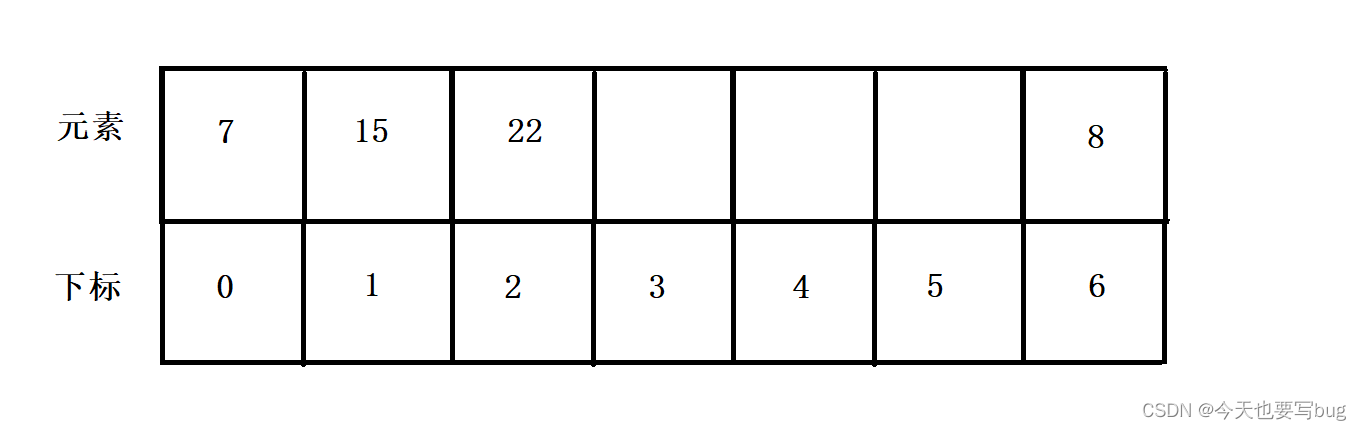🍏 注意❗️ ❗️

🌳🌳 二次探测可以防止成堆的哈希冲突都连续存储，缓解了效率的下降。

### 🌱 开散列

🌵🌵开散列也叫做 拉链法、哈希桶。首先对关键码集合用散列函数计算散列地址，具有相同地址的关键码归于同一子集合，每一个子集合称为一个桶，各个桶中的元素通过一个单链表链接起来，各链表的头结点存储在哈希表中。其本质就是一个指针数组，数组中存储的是单链表的首元素地址。

还是这个数组：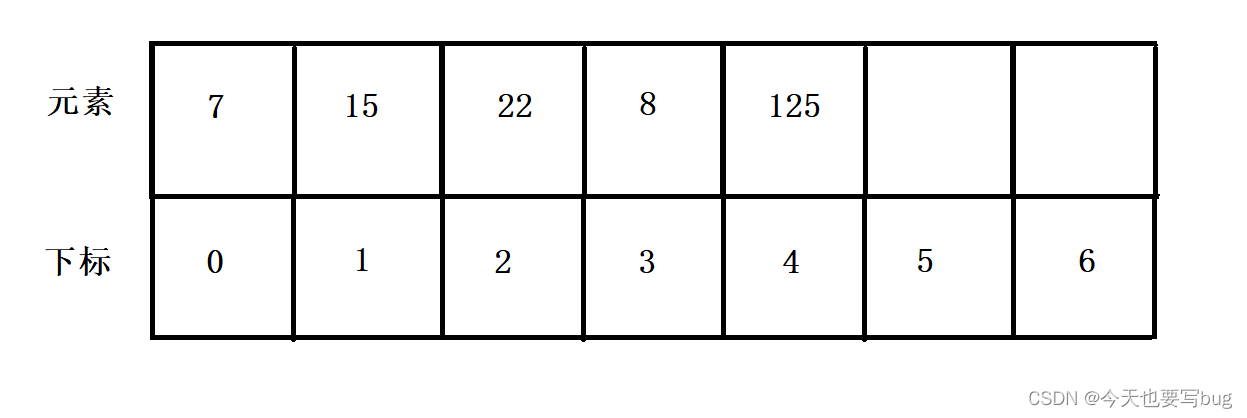我们要将它插入哈希表（开散列）中，如下图：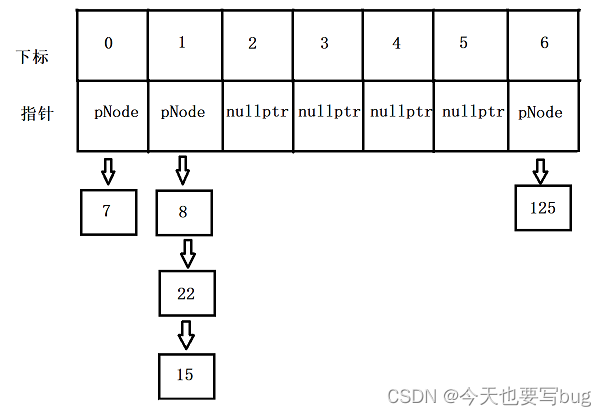当我们插入15、22和8时，取模计算位置，然后采用头插的方式，直接插入。发生哈希冲突的元素就通过链表进行存储。

# 二、闭散列（哈希）的模拟实现

🌻 🌻问题一：哈希表内部有什么结构？
（迭代器+哈希结点+哈希表。）

迭代器正向迭代器内部成员有结点指针+哈希表的指针，因为结点和结点之间没有联系，我们要从一个结点跳转到下一个结点就要通过哈希表来进行调整。所以需要哈希表的指针，同时传参过程可以很巧妙地利用this指针完成。反向迭代器通过封装正向迭代器来实现，闭散列的哈希表可以完成自减的重载，所以反向迭代器可以实现。
哈希结点成员变量有data数据，state状态。
哈希表封装哈希结点和迭代器于一体。

🌻 🌻 问题二：当我们插入删除数据时，怎么判断当前位置是否可以操作？
使用一个枚举类型，里面包含EMPTY、EXITS、DELETE三种状态。默认状态为EMPTY，插入数据后状态变为EXITS，删除数据时，只要把状态改成DELETE即可。

🌻 🌻 是不是会觉得很困惑，为什么存在三种状态？DELETE有什么作用呢？

假设有一个哈希表，如下图：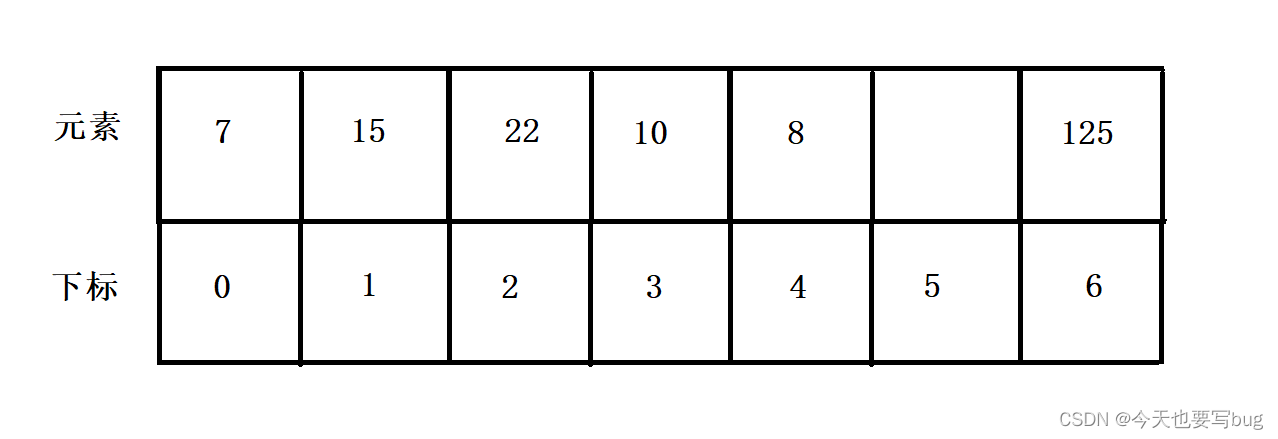当我们删除数据22时，将其置为EMPTY，但是会影响查找过程。查找时先计算数据的下标，如果当前位置数据不是查找的数据，那么向后依次查找，直到查到当前位置被标记为EMPTY时停止。在我们查找的过程中，如果删除数据就将其置EMPTY，当发生扎堆的哈希冲突，删除冲突中间的一个数据，就会影响对后面冲突数据的查询，所以要用DELETE进行标识，这样不会影响查找过程。如下图：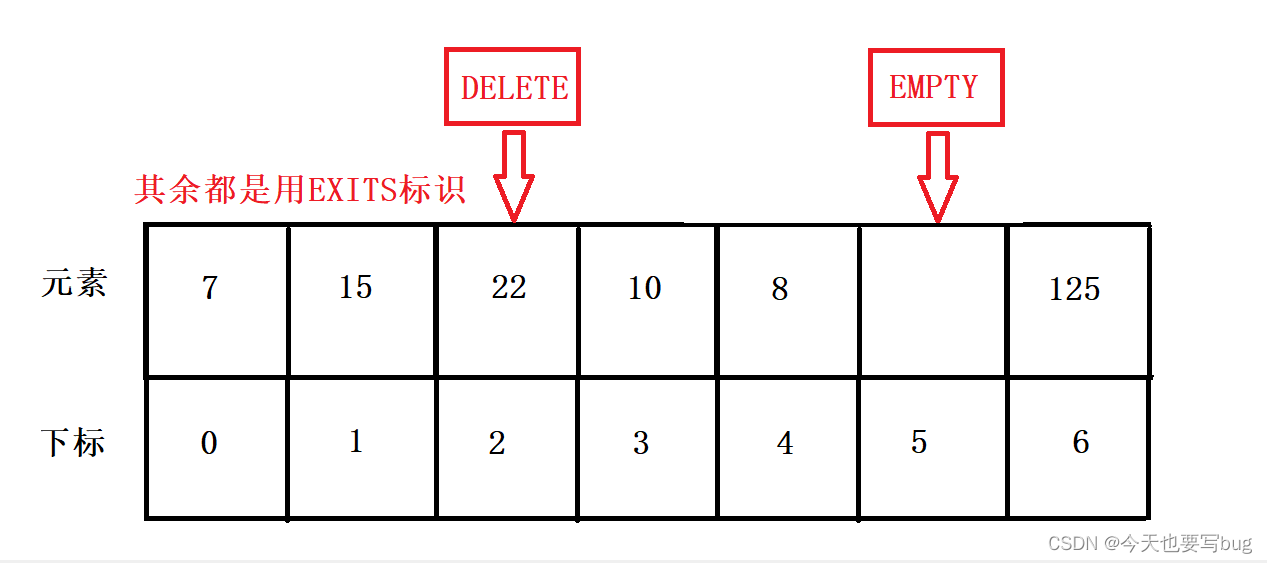当我们查找8的时候，计算下标为1 。但是如果22被标记成EMPTY，那么查找到22这个位置时就停止，但是如果是DELETE，那么会继续向后面查找。

🌻 🌻 问题三：怎么控制哈希表的大小？
当我们的表过小时，会产生大量的哈希冲突，效率会大幅度下滑。但是当我们的表过大时，哈希冲突得到了缓解，但是大量的空间又会被浪费。所以这里我们引入负载因子，负载因子即：有效的数据的数量/哈希表的长度，当负载因子超过0.7的时候，我们就要给哈希表扩容。为什么选中0.7作为标准值呢？在0.7的时候，哈希冲突和空间浪费之间得到了平衡，算的上是比较好的选择了。
当我们对哈希表进行扩容后，映射关系要进行调整。因为扩容的本质是重新开辟更大的空间，再将数据拷贝过去。但是，当哈希表扩容，其映射关系也会发生改变。所以我们不能直接将数据原封不动的拷贝过去，而是要重新建立映射关系。
在VS的编译器里，其采用了 素数表。因为按照素数进行扩容，取模计算下标时不容易发生哈希冲突。（虽然我也不太清楚怎么计算的）

🌻 🌻问题四：哈希表有K模型和KV模型，我们如何实现呢？
采用仿函数来取pair键对中的key，即如果是K模型，那么直接返回；如果是键对模型，那么选出里面的key再返回。

🌻 🌻问题五：字符串（比较常用）通过什么方式进行转化可以更好的避免哈希冲突呢？
（这里我们也要采用仿函数来实现。）

（不可取）
（1）首字符的ACSII码
字符总共只有256个，如果只用首字符来映射的话，很容易产生哈希冲突。
（2）字符串内所有字符ASCII码值之和
所有字符ACSII码值之和也容易冲突，即字符串中的字符相同，但是顺序不同时，全部都会冲突。

对于字符串，一群大佬经过研究后得出了下面几种不容易冲突的算法：

🍋 BKDR哈希算法⬇️ ⬇️ :

hash = hash * 131 + ch;   // 也可以乘以31、131、1313、13131、131313..


🍋 SDBM哈希算法⬇️ ⬇️ :

hash = 65599 * hash + ch;


🍋 RS哈希算法⬇️ ⬇️ ：

template<class T>
size_t RSHash(const T *str)
{
register size_t hash = 0;
size_t magic = 63689;
while (size_t ch = (size_t)*str++)
{
hash = hash * magic + ch;
magic *= 378551;
}
return hash;
}


（剩下的就不介绍了，有兴趣的可以去看看。网址： 哈希算法

🌻 🌻问题六：查找中怎么判断当前数据是否是我们需要的查找内容？
如果是内置类型，直接用等号比较即可。但是如果是自定义类型，它的比较就需要我们自己来完成。例如：字符串的比较，我们可以用string自己的等号重载来判断。所以采用仿函数来完成这项内容，需要比较自定义类型，那么就自己来写仿函数进行判断。

🌻 🌻 问题七：方括号（“[]”）的重载？
在KV模型中，方括号的重载是很重要的。即可以直接使用方括号进行插入数据，或者对数据进行修改。
进行重载中，调用insert函数进行插入，insert函数的返回是键对值。键对值中第一个参数迭代器类型，是插入位置的迭代器；第二个参数是布尔类型，判断插入是否成功。我们根据返回值中的迭代器参数可以获得插入位置的指针，然后再通过指针获得插入键对值中的V（这里插入过程中，V值为默认值），将V返回后，我们可以通过等号进行修改
⬇️ ⬇️

	V& operator[](const K& key)
{
return (insert(std::make_pair(key, V())).first._pnode->_data).second;
}

//使用方式
HTs["look"] = "看";
HTs.operator[]("eat") = "吃";


🌻 🌻其他问题：
因为迭代器中要使用哈希表的指针，而哈希表又要使用迭代器，所以我们需要添加一个前置声明。
迭代器中需要访问哈希表的私有成员_table，所以我们要把迭代器定义为哈希表的友元类。（不到万不得已，不使用友元，因为友元会破坏封装）

🍒 🍒 HashTable（闭散列）的完整代码⬇️ ⬇️ ：

#pragma once
#include<iostream>
#include<vector>
#include<string>

using std::cin;
using std::cout;
using std::endl;
using std::vector;
using std::string;
using std::pair;
using std::make_pair;

//选出key
template<class K, class V>
struct PairSelect1st
{
const K& operator()(const pair<K, V>& kv) { return kv.first; }
};
template<class K>
struct KSelect1st
{
const K& operator()(const K& k) { return k; }
};

//转成整型
template<class K>
struct HashFunc
{
size_t operator()(const K& val) { return val; }
};
//模板的特化
template<>
struct HashFunc<std::string>
{
size_t operator()(const std::string& s1)
{
size_t sum = 0;
for (size_t i = 0; i < s1.size(); i++)
{
sum = sum * 131 + s1[i];
}

return sum;
}
};

//比较判断
template<class K>
struct equal_to
{
bool operator()(const K& lval, const K& rval) { return lval == rval; }
};
template<>
//模板特化
struct equal_to<std::string>
{
bool operator()(const std::string& s1, const  std::string& s2) { return s1 == s2; }
};

//素数表
const int PRIMECOUNT = 28;
const size_t primeList[PRIMECOUNT] = {
53ul, 97ul, 193ul, 389ul, 769ul,
1543ul, 3079ul, 6151ul, 12289ul, 24593ul,
49157ul, 98317ul, 196613ul, 393241ul, 786433ul,
1572869ul, 3145739ul, 6291469ul, 12582917ul, 25165843ul,
50331653ul, 100663319ul, 201326611ul, 402653189ul, 805306457ul,
1610612741ul, 3221225473ul, 4294967291ul
};

namespace CloseHash
{
enum State { EMPTY, EXITS, DELETE };

template<class T>
struct HashData
{
T _data;//存储可能是k，可能是kv
State _state = EMPTY;//标识状态

HashData(const T& data = T(), State state = EMPTY) :_data(data), _state(state) {}
};

template<class K, class V, class T, class Pred, class Select1st, class HashFunc>
class HashTable;

template<class K, class V, class T, class Ref, class Ptr, class Pred = equal_to<K>
, class Select1st = PairSelect1st<K, V>, class HashFunc = HashFunc<K>>
struct Iterator
{
typedef HashData<T> HD;
typedef HashTable<K, V, T, Pred, Select1st, HashFunc> HT;
typedef Iterator<K, V, T, Ref, Ptr, Pred, Select1st, HashFunc> self;
typedef Ref reference;
typedef Ptr pointer;
HD* _pnode;
HT* _pht;

Iterator(HD* pnode = nullptr, HT* pht = nullptr) :_pnode(pnode), _pht(pht) {  }

bool operator!=(const self& it)const { return _pnode != it._pnode; }
bool operator==(const self& it)const { return _pnode == it._pnode; }

Ref operator*() { return _pnode->_data; }
Ptr operator->() { return &_pnode->_data; }
self& operator++()
{
size_t index = HashFunc()(Select1st()(_pnode->_data)) % _pht->_table.size();
HD* cur = &_pht->_table[index];
//找到_pnode的位置
while (_pnode != cur)
{
index++;
//如果到尾没找到，就从头开始找
if (index >= _pht->_table.size())
index = 0;

cur = &_pht->_table[index];
if (_pnode == cur)
break;
}

index++;
if (index >= _pht->_table.size())
{
_pnode = nullptr;
return *this;
}
//去找_pnode后面的一个数据
cur = &_pht->_table[index];
while (cur->_state != EXITS)
{
index++;
//如果下标不小于_table的大小时，遍历结束
if (index >= _pht->_table.size())
{
_pnode = nullptr;
return *this;
}
cur = &_pht->_table[index];
}

_pnode = cur;
return *this;
}
self operator++(int) { self tmp = *this; ++*this; return tmp; }

self& operator--()
{
int index = HashFunc()(Select1st()(_pnode->_data)) % _pht->_table.size();
HD* cur = &_pht->_table[index];
//找到_pnode的位置
while (_pnode != cur)
{
index++;
if (index >= _pht->_table.size())
index = 0;

cur = &_pht->_table[index];
if (_pnode == cur)
break;
}

index--;
if (index < 0)
{
_pnode = nullptr;
return *this;
}
//去找_pnode前面的一个数据
cur = &_pht->_table[index];
while (cur->_state != EXITS)
{
index--;
//如果index小于0，那么就置空返回。
if (index < 0)
{
_pnode = nullptr;
return *this;
}
cur = &_pht->_table[index];
}
_pnode = cur;
return *this;
}
self operator--(int) { self tmp = *this; --(*this); return tmp; }
};

template<class iterator>
struct Reverse_Iterator
{
typedef const iterator const_reverse_iterator;
typedef typename iterator::reference Ref;
typedef typename iterator::pointer Ptr;
typedef Reverse_Iterator self;
iterator _it;

Reverse_Iterator(iterator it) :_it(it) {}

Ref operator*() { return *_it; }
Ptr operator->() { return &(*_it) ; }
self& operator++() { _it--; return *this; }
self operator++(int) { self tmp = *this; _it--; return tmp; }
bool operator ==(const self& it) { return _it._pnode == it._it._pnode; }
bool operator != (const self& it) { return _it._pnode != it._it._pnode; }
};

template
<class K,class V,class T,class Pred = equal_to<K>
,class Select1st = PairSelect1st<K, V>, class HashFunc = HashFunc<K>>
class HashTable
//参数有KVT（键对值），仿函数选择键对值的key，仿函数将非整型转换成整型,仿函数进行比较
{
template<class K, class V, class T, class Ref, class Ptr, class Pred, class Select1st, class >
friend struct Iterator;
typedef HashData<T> HD;
typedef HashTable<K, V, T, Pred, Select1st, HashFunc> HT;
public:
typedef Iterator<K, V, T, const T&, const T*, Pred, Select1st, HashFunc> const_iterator;
typedef Iterator<K, V, T, T&, T *, Pred, Select1st, HashFunc> iterator;
typedef Reverse_Iterator<const_iterator> const_reverse_iterator;
typedef Reverse_Iterator<iterator> reverse_iterator;

private:
vector<HD> _table;
size_t _n;//记录有效数据的个数
public:
HashTable() :_n(0) { }

HashTable(const HT& h)
{
for (auto& e : h._table)
{
if(e._state == EXITS)
insert(e._data);
}
_n = h._n;
}

HT& operator=(const HT& h) { HT tmp(h); swap(tmp); return *this; }

iterator begin()
{
//如果迭代器为空直接返回
if (_n == 0)
return iterator(nullptr, this);
size_t index = 0;
HD* cur = &_table[index];
while (cur->_state != EXITS)
{
index++;
if (index >= _table.size())
return iterator(nullptr, this);
cur = &_table[index];
}
return iterator(cur, this);
}
iterator end() { return iterator(nullptr, this); }

const_iterator cbegin()
{
//如果迭代器为空直接返回
if (_n == 0)
return const_iterator(nullptr, this);
size_t index = 0;
HD* cur = &_table[index];
while (cur->_state != EXITS)
{
index++;
if (index >= _table.size())
return const_iterator(nullptr, this);
cur = &_table[index];
}
return const_iterator(cur, this);
}
const_iterator cend() { return const_iterator(nullptr, this); }

reverse_iterator rbegin()
{
//如果迭代器为空直接返回
if (_n == 0)
return reverse_iterator(iterator(nullptr, this));
size_t index = _table.size() - 1;
HD* cur = &_table[index];
while (cur->_state != EXITS)
{
index--;
if (index < 0)
return reverse_iterator(iterator(nullptr, this));
cur = &_table[index];
}
return reverse_iterator(iterator(cur, this));
}
reverse_iterator rend() { return reverse_iterator(iterator(nullptr, this)); }

const_reverse_iterator crbegin()
{
//如果迭代器为空直接返回
if(_n == 0)
return const_reverse_iterator(const_iterator(nullptr, this));

size_t index = _table.size() - 1;
HD* cur = &_table[index];
while (cur->_state != EXITS)
{
index--;
if (index < 0)
return const_reverse_iterator(const_iterator(nullptr, this));
cur = &_table[index];
}
return const_reverse_iterator(const_iterator(cur, this));
}
const_reverse_iterator crend() { return const_reverse_iterator(const_iterator(nullptr, this)); }

void swap(HT& h)
{
_table.swap(h._table);
std::swap(_n, h._n);
}

V& operator[](const K& key) { return (insert(std::make_pair(key, V())).first._pnode->_data).second; }

pair<iterator,bool> insert(const T& data)
{
//仿函数挑选
Select1st st1;
//仿函数转化
HashFunc hf;

if (!_table.size())
_table.resize(53ul);

//先判断是否key重复
iterator ret = find(data);
if (ret._pnode != nullptr)
return std::make_pair(ret,false);

//负载因子调节表的大小
if ((double)_n  / (double) _table.size() >= 0.7)
{
HT tmp;
tmp._table.resize(GetNextPrime(_table.size()));
auto it = _table.begin();
while (it != _table.end())
{
if (it->_state == EXITS)
tmp.insert(it->_data);
it++;
}
_table.swap(tmp._table);
}

//插入过程
size_t i = 1;//探测的数据
size_t index = (hf(st1(data))) % _table.size();//下标计算
while (_table[index]._state == EXITS)
{
//线性探测
index += i;
//二次探测
//index += i * i;
//i++;
if (index >= _table.size())
index %= _table.size();
}

_table[index]._data = data;
_table[index]._state = EXITS;
_n++;
return std::make_pair(iterator(& _table[index],this),true);
}

iterator find(const T& data)
{
Select1st slt;
HashFunc hf;
size_t index = hf(slt(data)) % _table.size();

//相等为真,这里需要先判断一次，再进循环
if (Pred()(slt(_table[index]._data), slt(data)))
return iterator(&_table[index], this);

while(1)
{
//相等为真
if (Pred()(slt(_table[index]._data), slt(data)))
return iterator(&_table[index], this);

if (_table[index]._state == EMPTY)
break;
index++;
//超过了,就取模
if (index >= _table.size())
index %= _table.size();
}

return iterator(nullptr,this);
}

bool erase(const T& data)
{
if (!empty())
{
iterator ret = find(data);
if (ret._pnode != nullptr)
{
ret._pnode->_state = DELETE;
_n--;
return true;
}
}
return false;
}
//获得素数
size_t GetNextPrime(size_t prime)
{
size_t i = 0;
for (; i < PRIMECOUNT; i++)
{
if (primeList[i] > prime)
return primeList[i];
}

return primeList[i];
}

bool empty()const { return _n == 0; }
size_t size()const { return _n; }
};
}



🍒 🍒 测试代码⬇️ ⬇️

//CloseHash(闭散列)
void Test_KV1()//KV模型：字典
{
CloseHash::HashTable
<string, string, pair<string, string>, equal_to<string>,PairSelect1st<string, string>> HTs;

pair<string, string> arr[] = {
make_pair("left", "左边") ,make_pair("right", "右边"),make_pair("up", "向上")
,make_pair("down", "向下"),make_pair("left","左边"),make_pair("eat","吃")
,make_pair("sleep","睡觉"),make_pair("run","跑"),make_pair("jump","跳") };
for (auto e : arr)
HTs.insert(e);

//方括号测试
HTs["look"] = "看";

//非const迭代器
CloseHash::HashTable<string, string, pair<string, string>
, equal_to<string>, PairSelect1st<string, string>>::iterator it1 = HTs.begin();
while (it1 != HTs.end())
{
cout << it1->first << ":" << it1->second << endl;
it1++;
}
cout << endl;

//反向迭代器
auto rit1 = HTs.rbegin();
while (rit1 != HTs.rend())
{
cout << rit1->first << ":" << rit1->second << endl;
rit1++;
}
cout << endl;

//删除测试
HTs.erase(make_pair("left", "左边"));
HTs.erase(make_pair("left", "左边"));
HTs.erase(make_pair("left", "左边"));
HTs.erase(make_pair("down", "向下"));

//const迭代器
CloseHash::HashTable<string, string, pair<string, string>
, equal_to<string>, PairSelect1st<string, string>>::const_reverse_iterator crit1 = HTs.crbegin();
while (crit1 != HTs.crend())
{
cout << crit1->first << ":" << crit1->second << endl;
crit1++;
}
cout << endl;
}

void Test_K1()//K模型
{
CloseHash::HashTable<string, string, string, equal_to<string>, KSelect1st<string>>HTs;
string arr[] = {
"left", "左边" ,"right", "右边","up", "向上"
,"down", "向下","left","左边","eat","吃"
,"sleep","睡觉","run","跑","jump","跳" };
for (auto e : arr)
HTs.insert(e);

//非const迭代器
CloseHash::HashTable<string, string, string,
equal_to<string>, KSelect1st<string>>::iterator it = HTs.begin();
while (it != HTs.end())
{
cout << *it << endl;
it++;
}
cout << endl;

//删除功能
HTs.erase("r");
HTs.erase("up");
HTs.erase("up");
HTs.erase("dowm");

//const迭代器
auto cit = HTs.cbegin();
while (cit != HTs.cend())
{
cout << *cit << endl;
cit++;
}
cout << endl;
}


# 三、开散列（哈希）的模拟实现

开散列中许多内容和闭散列一致，这里我们主要谈谈它的区别：

🌻 🌻 问题一：开散列需不需要状态来进行标识？
开散列不需要标识，插入发生哈希冲突时，直接头插即可。删除结点，如果只有一个结点，直接释放后，将_table中的指针置空。如果有多个结点，释放结点后，直接将前一个结点和后一个结点相连。查找也只需要计算下标后，找到对应的桶，然后进行查找。

🌻 🌻 问题二：开散列的迭代器如何实现？
和闭散列的迭代器类似，即需要结点指针和哈希表的指针。遍历过程就是一个一个桶进行遍历，桶内遍历直接根据next指针即可。桶间的转换需要借助哈希表的指针。因为我们的哈希表采用的是vector+forward_list，所以不能够重载自减，即无法实现反向迭代器。（单链表不支持，双向链表可以支持）

🌻 🌻 问题三：迭代器中的自增如何重载？
先计算当前结点指针处于哪一个桶中，然后去桶中遍历，直到找到当前结点指针，然后再判断，如果为尾，那么我们就去找下一个桶，直到找到存储数据的桶，然后将我们的结点指针修改成桶中的第一个指针。

🌻 🌻 问题四：极端情况应该怎么解决？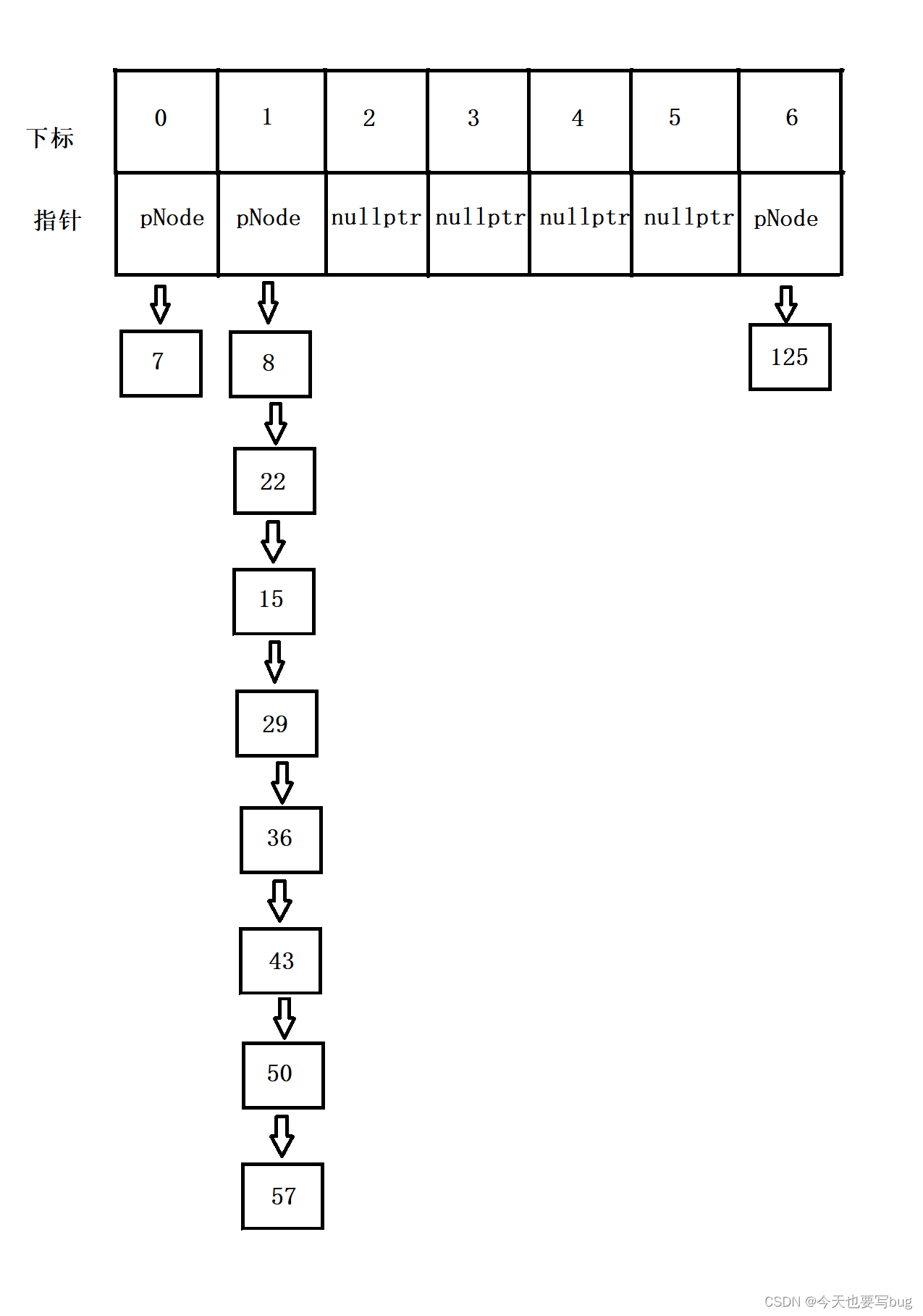极端情况发生的概率极低，而且发生之后即使效率大幅度降低也只是暂时的，只要继续插入数据，效率又会恢复。但是在java中对这种情况还是进行了处理，即每次插入数据之后都会统计链表的长度，如果链表中的结点个数达到了8个，那么就会将单链表转化成红黑树来进行存储。如果发生扩容，即重新映射后，结点的个数小于8，那么又会转化成单链表进行存储。

🌻 🌻问题五：开散列的平衡因子该怎么来控制？
在开散列中其平衡因子设置为达到1时，进行扩容。即数据个数等于桶的个数。在开散列中，我们不用去担心数据的插入问题，因为不论多少个数据一定都可以插入，因为数据存储在链表中。但是当链表的长度过长时，其操作效率会大幅度下降，所以我们要控制好扩容时机，不能浪费过多的空间，同时也不能使效率下降的过多。

🍒 🍒 HashTable（开散列）的完整代码⬇️ ⬇️

#pragma once
#include<iostream>
#include<vector>
#include<string>

using std::cin;
using std::cout;
using std::endl;
using std::vector;
using std::string;
using std::pair;
using std::make_pair;

//选出key
template<class K, class V>
struct PairSelect1st
{
const K& operator()(const pair<K, V>& kv) { return kv.first; }
};
template<class K>
struct KSelect1st
{
const K& operator()(const K& k) { return k; }
};

//转成整型
template<class K>
struct HashFunc
{
size_t operator()(const K& val) { return val; }
};
//模板的特化
template<>
struct HashFunc<std::string>
{
size_t operator()(const std::string& s1)
{
size_t sum = 0;
for (size_t i = 0; i < s1.size(); i++)
{
sum = sum * 131 + s1[i];
}

return sum;
}
};

//比较判断
template<class K>
struct equal_to
{
bool operator()(const K& lval, const K& rval) { return lval == rval; }
};
template<>
//模板特化
struct equal_to<std::string>
{
bool operator()(const std::string& s1, const  std::string& s2) { return s1 == s2; }
};

//素数表
const int PRIMECOUNT = 28;
const size_t primeList[PRIMECOUNT] = {
53ul, 97ul, 193ul, 389ul, 769ul,
1543ul, 3079ul, 6151ul, 12289ul, 24593ul,
49157ul, 98317ul, 196613ul, 393241ul, 786433ul,
1572869ul, 3145739ul, 6291469ul, 12582917ul, 25165843ul,
50331653ul, 100663319ul, 201326611ul, 402653189ul, 805306457ul,
1610612741ul, 3221225473ul, 4294967291ul
};
namespace OpenHash
{
template<class T>
struct HashNode
{
typedef HashNode<T> Node;
typedef HashNode<T>* pNode;

HashNode<T>* _next;
T _data;

public:
HashNode(const T& data = T())
:_next(nullptr)
,_data(data)
{}
};

template<class K, class V, class T, class Pred, class Select1st ,class HashFunc>
class HashTable;

template<class K, class V, class T, class Ref, class Ptr, class Pred, class Select1st, class HashFunc>
struct Iterator
{
typedef HashNode<T> Node;
typedef HashTable<K, V, T, Pred, Select1st, HashFunc> HashTable;
typedef Iterator<K, V, T, Ref, Ptr, Pred, Select1st, HashFunc> self;

Node* _pnode;
HashTable* _pHT;

Iterator(Node* pnode = nullptr, HashTable* pHT = nullptr) : _pnode(pnode), _pHT(pHT) {  }

Ref operator*() { return _pnode->_data; }
const Ref operator*()const { return _pnode->_data; }
Ptr operator->() { return &_pnode->_data; }
const Ptr operator->()const { return &_pnode->_data; }

self& operator++()
{
if (_pnode == nullptr)
return *this;

if (_pnode->_next != nullptr)
{
_pnode = _pnode->_next;
return *this;
}

//_pnode->next == nullptr我们要去找现在的结点属于哪一个桶
size_t index = HashFunc()(Select1st()(_pnode->_data)) % _pHT->_table.size() + 1;
for (; index < _pHT->_table.size(); index++)
{
Node* cur = _pHT->_table[index];
if (cur != nullptr)
{
_pnode = cur;
return *this;
}
}
_pnode = nullptr;
return *this;
}
self operator++(int)
{
self tmp = *this;
++(*this);
return tmp;
}

bool operator!=(const self& it)const { return _pnode != it._pnode; }
bool operator==(const self& it)const { return _pnode == it._pnode; }
};

template
<class K, class V, class T, class Pred = equal_to<std::string>,
class Select1st = PairSelect1st<K, V>, class HashFunc = HashFunc<K>>
class HashTable
{
typedef HashNode<T>* pNode;
typedef HashNode<T> Node;

template<class K, class V, class T, class Ref, class Ptr, class Pred, class Select1st, class HashFunc>
friend  struct Iterator;
private:
//存结点指针
vector<pNode> _table;
size_t _n;
public:
typedef Iterator<K, V, T, const T&, const T* ,Pred, Select1st, HashFunc> const_iterator;
typedef Iterator<K, V, T, T&, T*, Pred, Select1st, HashFunc> iterator;

HashTable() :_n(0) {  }

void clear()
{
for (size_t index = 0; index < _table.size(); index++)
{
pNode cur = _table[index];
pNode prev = cur;
while (cur)
{
prev = cur;
cur = cur->_next;
delete prev;
}
}
}

~HashTable()
{
clear();
}

iterator begin()
{
size_t index = 0;
for (; index < _table.size(); index++)
{
pNode cur = _table[index];
if (cur != nullptr)
return iterator(cur,this);
}
return iterator(nullptr, this);
}
iterator end() { return iterator(nullptr, this); }

const_iterator cbegin()
{
size_t index = 0;
for (; index < _table.size(); index++)
{
pNode cur = _table[index];
if (cur != nullptr)
return const_iterator(cur, this);
}
return const_iterator(nullptr, this);
}
const_iterator cend() { return const_iterator(nullptr, this); }

pair<iterator,bool> insert(const T& data)
{
//如果为空，则开空间
if (!_table.size())
_table.resize(53ul);
//挑选key
Select1st st1;
//转换整型
HashFunc hf;
//判断是否冗余
iterator ret = find(data);
if (ret._pnode != nullptr)
return std::make_pair(iterator(nullptr,this), false);

//判断是否需要扩容
if ((double)_n / (double)_table.size() >= 1)
{
vector<pNode> new_table(GetNextPrime(_table.size()));
for (size_t i = 0; i < _table.size(); i++)
{
pNode cur = _table[i];
if (cur != nullptr)
{
pNode next = _table[i];
while (cur)
{
next = cur->_next;
size_t new_index = (hf(st1(cur->_data))) % new_table.size();
//头插
cur->_next = new_table[new_index];
new_table[new_index] = cur;
cur = next;
}
_table[i] = nullptr;
}
//不推荐，插入的时候重新创建结点，浪费
/*while(e != nullptr)
{
tmp.insert(e->_kv);
e = e->_next;
}*/
}
new_table.swap(_table);
}

//计算hashbucket的下标
size_t index = hf(st1(data)) % _table.size();
pNode newNode = new  Node(data);
//头插
newNode->_next = _table[index];
_table[index] = newNode;
_n++;

return std::make_pair(iterator(newNode,this), true);
}

iterator find(const T& data)
{
HashFunc hf;
Select1st slt;
if (_table.size() == 0)
return iterator(nullptr,this);

size_t index = hf(slt(data)) % _table.size();
pNode cur = _table[index];
while (cur)
{
if (Pred()(slt(cur->_data), slt(data)))
return iterator(cur,this);
else
cur = cur->_next;
}

return iterator(nullptr,this);
}

bool erase(const T& data)
{
Select1st st1;
size_t index = HashFunc()(st1(data)) % _table.size();
pNode cur = _table[index];
pNode prev = cur;
while (cur)
{
if (Pred()(st1(cur->_data) , st1(data)))
{
//找到了
if (cur == _table[index])//头结点
{
_table[index] = nullptr;
_n--;
delete cur;
return true;
}
else
{
prev->_next = cur->_next;
_n--;
delete cur;
return true;
}
}
else//没找到
{
prev = cur;
cur = cur->_next;
}
}
return false;
}

size_t GetNextPrime(size_t prime)
{
size_t i = 0;
for (; i < PRIMECOUNT; i++)
{
if (primeList[i] > primeList[prime])
return primeList[i];
}

return primeList[i];
}

size_t size() const{ return _n; }
};
}


🍒 🍒 测试代码⬇️ ⬇️

//OpenHash(开散列)
void Test_KV2()//KV模型
{
OpenHash::HashTable<string, string, pair<string, string>> hts;

pair<string, string> arr[] = {
make_pair("left", "左边") ,make_pair("right", "右边"),make_pair("up", "向上")
,make_pair("down", "向下"),make_pair("left","左边"),make_pair("eat","吃")
,make_pair("sleep","睡觉"),make_pair("run","跑"),make_pair("jump","跳")};

for (auto e : arr)
hts.insert(e);

//非const迭代器
OpenHash::HashTable<string, string, pair<string, string>>::iterator it = hts.begin();
while (it != hts.end())
{
cout << it->first << ":" << it->second << endl;
it++;
}
cout << endl;

hts.erase(make_pair("sleep", "睡觉"));
hts.erase(make_pair("left", "左边"));
hts.erase(make_pair("up", "向上"));

//const类型
OpenHash::HashTable<string, string, pair<string, string>>::const_iterator cit = hts.cbegin();
while (cit != hts.cend())
{
cout << cit->first << ":" << cit->second << endl;
cit++;
}
cout << endl;
}

void Test_K2()//K模型
{
OpenHash::HashTable<string, string, string, equal_to<string>, KSelect1st<string>, HashFunc<string>> hts;
string arr[] = {
"left", "左边" ,"right", "右边","up", "向上"
,"down", "向下","left","左边","eat","吃"
,"sleep","睡觉","run","跑","jump","跳" };
for (auto e : arr)
hts.insert(e);

OpenHash::HashTable<string, string, string, equal_to<string>, KSelect1st<string>, HashFunc<string>>::iterator it = hts.begin();
while (it != hts.end())
{
cout << *it << " ";
it++;
}
cout << endl;
}


🔍 🔍 总结：
空间消耗在开散列中虽然增加了链表的链接指针，但是其空间消耗还是要比闭散列小，因为闭散列的平衡因子一定会比开散列的小，闭散列的平衡因子一定小于1，而且越接近1，越容易发生哈希冲突。所以相同数量的数据进行存储时，开散列开辟的空间更小。

😎 😎 今天的内容到这里就结束了，希望小伙伴们能够有所收获。展开全文散列表 数据结构
• ## 哈希表C++简单实现

千次阅读 2019-02-28 09:16:59
1.什么是哈希表 哈希表（Hash table，也叫散列表），是根据关键码值(Key value)而直接进行访问的数据结构。也就是说，它通过把关键码值映射到表中一个位置来访问记录，以加快查找的速度。 1.1 哈希函数 也叫散列...数据结构和算法
• public成员包括构造函数、析构函数和复制构造函数以及=重载函数，其它成员函数主要有：traver(遍历散列表)、show()(打印出哈希表所存的元素)返回值为bool类型的函数search\insert\Delete。search函数（查询关键字为...
• 主要介绍了C++语言实现hash详解及实例代码的相关资料,需要的朋友可以参考下
• 主要介绍了C++ 实现哈希表的实例的相关资料,这里使用C++实现哈希表的实例帮助大家彻底理解哈希表的原理，需要的朋友可以参考下
• 哈希表 散列表,它是基于快速存取的角度设计的,也是一种典型的 “空间换时间” 的做法; 键(key) : 组员的编号, 如: 1,2,3…; 值(value): 组员的其他信息 (包含: 性别, 年龄, 战斗力等); 索引: 数组的下标 (0,1,2…), ...mysql c语言
• 设计哈希表 1.确定表的空间范围，确定哈希值域 2.构造哈希函数 （确保元素计算之后返回值在值域内） 3.处理冲突的办法（链式结构） 哈希函数 设计方法： 直接寻址法 -散列地址 键值对 数学分析法 -分析...散列表 数据结构
• 在刷初级算法的字符串有好多关于哈希表的内容 所以作词笔记，强化哈希。 首先哈希表的作用是什么？它可以快速的查找。key-value值。 key值是唯一的。就例如一个统计某几人的身高。 如下是STL标准库map容器的实例。...散列表
• C++实现哈希表创建、查找、显示、计算ALS（详细）。针对某个数值序列，设计一个哈希表，完成相应的建表和查表顺序。哈希函数用除留余数法构造，用线性探测再散列的方法处理哈希地址冲突。针对给定的数列：{23,5,17,...数据结构 算法
• ## 哈希表（C++实现）

千次阅读 2018-11-23 17:42:22
散列表能够实现通过key 对元素的快速访问。 而且易于扩展。 对元素能够实现快速访问（搜索等字典操作）， 这是Hash Table 较之于链表的优势所在， 二者均易于扩展。 而易于扩展这个dynamic的结构（使用链接法的时候...
• hash的简单实现 数据结构初学 对于这里希望留下一些资源 期待大家批评指正
• ## C++哈希表

千次阅读 多人点赞 2021-11-22 21:07:08
什么是哈希表 map、hash_map、unordered_map的引入 unordered_map的用法 1. 什么是哈希表 1.1 哈希表的定义 “散列表（Hash table，也叫哈希表），是根据键（Key）而直接访问在内存存储位置的数据结构。也就是说，...linux 运维 服务器
• 哈希表算法 hash表 问题描述 针对某个集体比如你所在的班级中的人名设计一个哈希表使得平均查找长度不超过R完成相应的建表和查表程序 基本要求 假设人名为中国人姓名的汉语拼音形式待填入哈希表的人名共有30个取平均...
• C++哈希表实现前言源码如下： 前言 本篇文章为笔者的读书笔记，未经允许请勿转载。如果对你有帮助记得点个赞(●’◡’●) 本文主要讲的哈希表的创建和使用， 源码如下： main #include <iostream> #...数据结构
• } /*遍历打印哈希表哈希表索引）*/ void print_hash(HASH_TABLE* hashtable_index) { int i = 0; if (NULL == hashtable_index) return; for (i = 0; i ; ++i) { NODE* tmp = hashtable_index->value[i]; while ...算法
• 设计哈希表实现图书查找系统，完成相应的建表和查表程序。从键盘输入各图书相关信息，分别以图书编号为关键字建立散列表。待填入哈希表的书号至少30个；构造合适的哈希函数。 （1）记录由外部输入。 （2）生成的哈希...
• 解决散列冲突文件结构字典类概念代码哈希类概念代码有序链表概念代码哈希表实现概念代码测试主函数代码输出 文件结构 字典类 概念 代码 //dictionary.h template<class K,class E> class dictionary { public:...数据结构 算法 散列表
• (seperate chaining )1、1实现1.2、测试2、哈希表的动态空间优化参考 前言 1、哈希表与哈希函数的引入 就像这道题来说，用一个26个的int 型数组，就可以实现对每个字符进行哈希映射。 其中哈希函数为：f(char) = ...数据结构 哈希函数
• 为什么会产生哈希的概念： 给一个常见的数组 { 501 ， 502 ， 503 ， 504 ， 505 ... 1000 } ，为了节省空间，一般采用通过相对映射的方式开辟空间，也就是开 500 的空间 ，0 对应 501 ，1 对应 502 ... ，如果要...
• 这个代码是我用C++编写的队列实现实现方式是数组的形式，对于刚学习数据结构的人来说里面的功能比较全面
• 在程序中我们对关键字key应用散列函数H(key)来判断关键字key是否在散列表中，...程序的具体实现如下：本程序是用模板类myhash来实现，包括protected和public属性成员。其中protected成员有*ht（自定义散列表指针）、*e
• //在这让size++的目的是为了让负载因子为一，即一个下下只挂一个数据 89 } 90 void show() 91 { 92 for(int i=0; i(); ++i) 93 { 94 cout第"个："; 95 table_node *cur=vector_node[i]; 96 while(cur !=......

# 哈希表c++实现c++ 订阅